# Everything you need to know about TensorFlow 2.0Everything you need to know about TensorFlow 2.0

On June 26 of 2019, I will be giving a TensorFlow (TF) 2.0 workshop at the PAPIs.io LATAM conference in São Paulo. Aside from the happiness of being representing Daitan as the workshop host, I am very happy to talk about TF 2.0.

The idea of the workshop is to highlight what has changed from the previous 1.x version of TF. In this text, you can follow along with the main topics we are going to discuss. And of course, have a look at the Colab notebook for practical code.

#### Introduction to TensorFlow 2.0

TensorFlow is a general purpose high-performance computing library open sourced by Google in 2015. Since the beginning, its main focus was to provide high-performance APIs for building Neural Networks (NNs). However, with the advance of time and interest by the Machine Learning (ML) community, the lib has grown to a full ML ecosystem.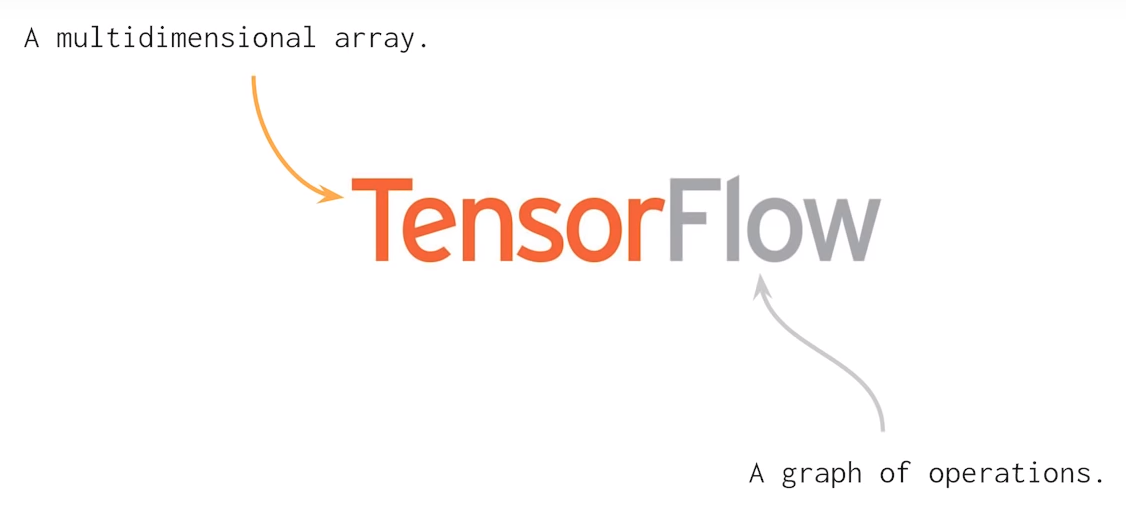Currently, the library is experiencing its largest set of changes since its birth. TensorFlow 2.0 is currently in beta and brings many changes compared to TF 1.x. Let’s dive into the main ones.

#### Eager Execution By Default

To start, eager execution is the default way of running TF code.

As you might recall, to build a Neural Net in TF 1.x, we needed to define this abstract data structure called a Graph. Also, (as you probably have tried), if we attempted to print one of the graph nodes, we would not see the values we were expecting. Instead, we would see a reference to the graph node. To actually, run the graph, we needed to use an encapsulation called a Session. And using the Session.run() method, we could pass Python data to the graph and actually train our models.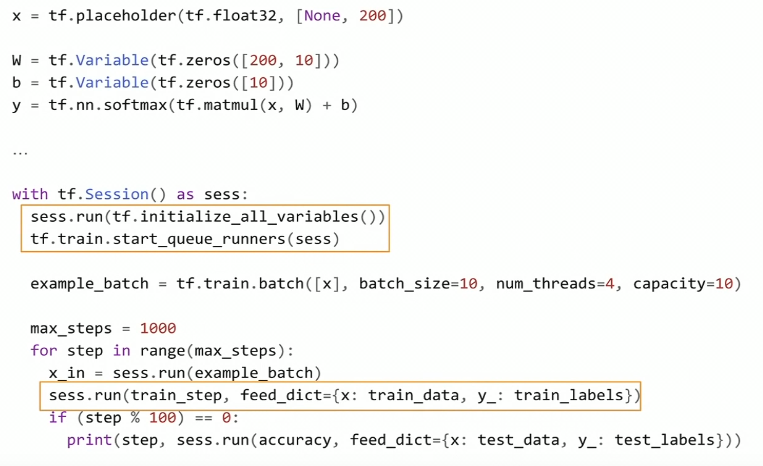With eager execution, this changes. Now, TensorFlow code can be run like normal Python code. Eagerly. Meaning that operations are created and evaluated at once.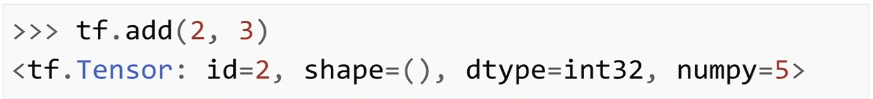TensorFlow 2.0 code looks a lot like NumPy code. In fact, TensorFlow and NumPy objects can easily be switched from one to the other. Hence, you do not need to worry about placeholders, Sessions, feed_dictionaties, etc.

#### API Cleanup

Many APIs like tf.gans, tf.app, tf.contrib, tf.flags are either gone or moved to separate repositories.

However, one of the most important cleanups relates to how we build models. You may remember that in TF 1.x we have many more than 1 or 2 different ways of building/training ML models.

Tf.slim, tf.layers, tf.contrib.layers, tf.keras are all possible APIs one can use to build NNs is TF 1.x. That not to include the Sequence to Sequence APIs in TF 1.x. And most of the time, it was not clear which one to choose for each situation.

Although many of these APIs have great features, they did not seem to converge to a common way of development. Moreover, if we trained a model in one of these APIs, it was not straight forward to reuse that code using the other ones.

In TF 2.0, tf.keras is the recommended high-level API.

As we will see, Keras API tries to address all possible use cases.

#### The Beginners API

From TF 1.x to 2.0, the beginner API did not change much. But now, Keras is the default and recommended high-level API. In summary, Keras is a set of layers that describes how to build neural networks using a clear standard. Basically, when we install TensorFlow using pip, we get the full Keras API plus some additional functionalities.

``````model = tf.keras.models.Sequential()

# Configures the model for training.
# Define the model optimizer, the loss function and the accuracy metrics
loss='sparse_categorical_crossentropy',
metrics=['accuracy'])
model.summary()
``````

sequencial.py

The beginner’s API is called Sequential. It basically defines a neural network as a stack of layers. Besides its simplicity, it has some advantages. Note that we define our model in terms of a data structure (a stack of layers). As a result, it minimizes the probability of making errors due to model definition.

#### Keras-Tuner

Keras-tuner is a dedicated library for hyper-parameter tuning of Keras models. As of this writing, the lib is in pre-alpha status but works fine on Colab with tf.keras and Tensorflow 2.0 beta.

It is a very simple concept. First, need to define a model building function that returns a compiled keras model. The function takes as input a parameter called hp. Using hp, we can define a range of candidate values that we can sample hyper-parameters values.

Below we build a simple model and optimize over 3 hyper-parameters. For the hidden units, we sample integer values between a pre-defined range. For dropout and learning rate, we choose at random, between some specified values.

``````def build_model(hp):
# define the hyper parameter ranges for the learning rate, dropout and hidden unit
hp_units = hp.Range('units', min_value=32, max_value=128, step=32)
hp_lr = hp.Choice('learning_rate', values=[1e-2, 1e-3, 1e-4])
hp_dropout = hp.Choice('dropout', values=[0.1,0.2,0.3])

# build a Sequential model
model = keras.Sequential()

# compile and return the model
loss='sparse_categorical_crossentropy',
metrics=['accuracy'])
return model

# create a Random Search tuner
tuner = RandomSearch(
build_model,
objective='val_accuracy', # define the metric to be optimized over
max_trials=3,
executions_per_trial=1,
directory='my_logs') # define the output log/checkpoints folder

# start hyper-parameter optmization search
tuner.search(x_train, y_train,
epochs=2,
validation_data=(x_test, y_test))
``````

keras-tuner.py

Then, we create a tuner object. In this case, it implements a Random Search Policy. Lastly, we can start optimization using the search() method. It has the same signature as fit().

In the end, we can check the tuner summary results and choose the best model(s). Note that training logs and model checkpoints are all saved in the directory folder (my_logs). Also, the choice of minimizing or maximizing the objective (validation accuracy) is automatically infered.

The moment you see this type of implementation it goes back to Object Oriented programming. Here, your model is a Python class that extends tf.keras.Model. Model subclassing is an idea inspired by Chainer and relates very much to how PyTorch defines models.

With model Subclassing, we define the model layers in the class constructor. And the call() method handles the definition and execution of the forward pass.

``````class Model(tf.keras.Model):
def __init__(self):
# Define the layers here
super(Model, self).__init__()
self.conv1 = Conv2D(filters=8, kernel_size=4, padding="same", strides=1, input_shape=(IMAGE_HEIGHT,IMAGE_WIDTH,IMAGE_DEPTH))
self.conv2 = Conv2D(filters=16, kernel_size=4, padding="same", strides=1)
self.flat = Flatten()
self.probs = Dense(units=N_CLASSES, activation='softmax', name="output")

def call(self, x):
# Define the forward pass
net = self.conv1(x)
net = self.pool(net)
net = self.conv2(net)
net = self.pool(net)
net = self.flat(net)
net = self.probs(net)
return net

def compute_output_shape(self, input_shape):
# You need to override this function if you want to use the subclassed model
# as part of a functional-style model.
# Otherwise, this method is optional.
shape = tf.TensorShape(input_shape).as_list()
shape[-1] = self.num_classes
return tf.TensorShape(shape)
``````

subclassing_model.py

Subclassing has many advantages. It is easier to perform a model inspection. We can, (using breakpoint debugging), stop at a given line and inspect the model’s activations or logits.

However, with great flexibility comes more bugs.

Model Subclassing requires more attention and knowledge from the programmer.

In general, your code is more prominent to errors (like model wiring).

#### Defining the Training Loop

The easiest way to train a model in TF 2.0 is by using the fit() method. fit() supports both types of models, Sequential and Subclassing. The only adjustment you need to do, if using model Subclassing, is to override the compute_output_shape() class method, otherwise, you can through it away. Other than that, you should be able to use fit() with either tf.data.Dataset or standard NumPy nd-arrays as input.

However, if you want a clear understanding of what is going on with the gradients or the loss, you can use the Gradient Tape. That is especially useful if you are doing research.

Using Gradient Tape, one can manually define each step of a training procedure. Each of the basic steps in training a neural net such as:

• Forward pass
• Loss function evaluation
• Backward pass

is separately specified.

This is much more intuitive if one wants to get a feel of how a Neural Net is trained. If you want to check the loss values w.r.t the model weights or the gradient vectors itself, you can just print them out.

Gradient Tape gives much more flexibility. But just like Subclassing vs Sequential, more flexibility comes with an extra cost. Compared to the fit() method, here we need to define a training loop manually. As a natural consequence, it makes the code more prominent to bugs and harder to debug. I believe that is a great trade off that works ideally for code engineers (looking for standardized code), compared to researchers who usually are interested in developing something new.

Also, using fit() we can easily setup TensorBoard as we see next.

#### Setting up TensorBoard

You can easily setup an instance of TensorBoard using the fit() method. It also works on Jupyter/Colab notebooks.

In this case, you add TensorBoard as a callback to the fit method.

As long as you are using the fit() method, it works on both: Sequential and the Subclassing APIs.

``````# Load the TensorBoard notebook extension

# create the tensorboard callback
tensorboard = TensorBoard(log_dir='logs/{}'.format(time.time()), histogram_freq=1)

# train the model
model.fit(x=x_train,
y=y_train,
epochs=2,
validation_data=(x_test, y_test),
callbacks=[tensorboard])

# launch TensorBoard
%tensorboard --logdir logs
``````

tensorboard.py

If you choose to use Model Subclassing and write the training loop yourself (using Grading Tape), you also need to define TensorBoard manually. It involves creating the summary files, using tf.summary.create_file_writer(), and specifying which variables you want to visualize.

As a worth noting point, there are many callbacks you can use. Some of the more useful ones are:

• EarlyStopping: As the name implies, it sets up a rule to stop training when a monitored quantity has stopped improving.
• ReduceLROnPlateau: Reduce the learning rate when a metric has stopped improving.
• TerminateOnNaN: Callback that terminates training when a NaN loss is encountered.
• LambdaCallback: Callback for creating simple, custom callbacks on-the-fly.

You can check the complete list at TensorFlow 2.0 callbacks.

#### Extracting Performance of your EagerCode

If you choose to train your model using Gradient Tape, you will notice a substantial decrease in performance.

Executing TF code eagerly is good for understanding, but it fails on performance. To avoid this problem, TF 2.0 introduces tf.function.

Basically, if you decorate a python function with tf.function, you are asking TensorFlow to take your function and convert it to a TF high-performance abstraction.

``````@tf.function
def train_step(images, labels):

# forward pass
predictions = model(images)

# compute the loss
loss = cross_entropy(tf.one_hot(labels, N_CLASSES), predictions)

# get the gradients w.r.t the model's weights

# perform a gradient descent step

# accumulates the training loss and accuracy
train_loss(loss)
train_accuracy(labels, predictions)
``````

tf_function.py

It means that the function will be marked for JIT compilation so that TensorFlow runs it as a graph. As a result, you get the performance benefits of TF 1.x (graphs) such as node pruning, kernel fusion, etc.

In short, the idea of TF 2.0 is that you can devise your code into smaller functions. Then, you can annotate the ones you wish using tf.function, to get this extra performance. It is best to decorate functions that represent the largest computing bottlenecks. These are usually the training loops or the model’s forward pass.

Note that when you decorate a function with tf.function, you loose some of the benefits of eager execution. In other words, you will not be able to setup breakpoints or use print() inside that section of code.

#### Save and Restore Models

Another great lack of standardization in TF 1.x is how we save/load trained models for production. TF 2.0 also tries to address this problem by defining a single API.

Instead of having many ways of saving models, TF 2.0 standardize to an abstraction called the SavedModel.

There is no much to say here. If you create a Sequential model or extend your class using tf.keras.Model, your class inherits from tf.train.Checkpoints. As a result, you can serialize your model to a SavedModel object.

``````# serialize your model to a SavedModel object
# It includes the entire graph, all variables and weights
model.save('/tmp/model', save_format='tf')

``````

gistfile1.py

SavedModels are integrated with the TensorFlow ecosystem. In other words, you will be able to deploy it to many different devices. These include mobile phones, edge devices, and servers.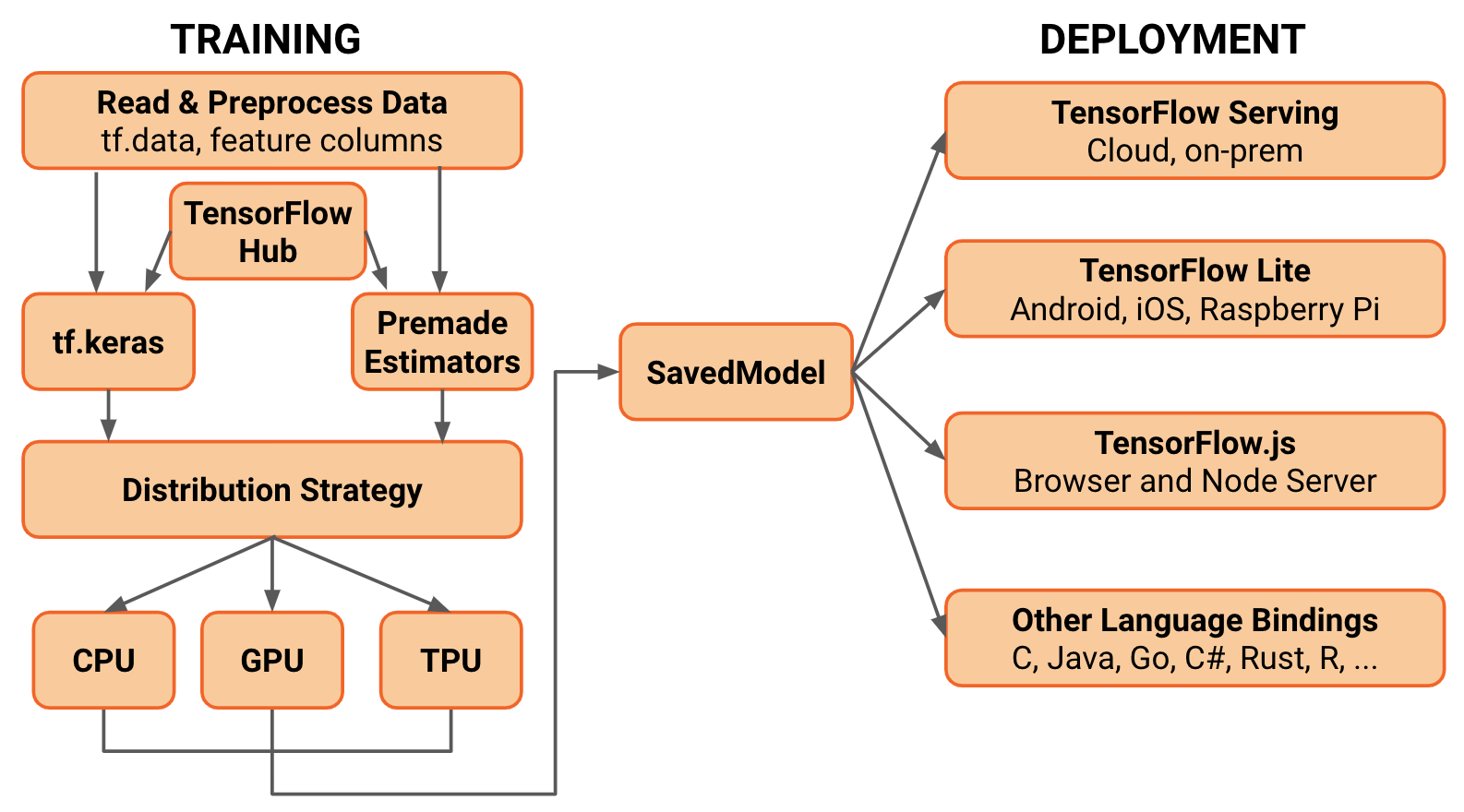#### Converting to TF-Lite

If you want to deploy a SavedModel to embedded devices like Raspberry Pi, Edge TPUs or your phone, use the TF Lite converter.

Note that in 2.0, the TFLiteConverter does not support frozen GraphDefs (usually generated in TF 1.x). If you want to convert a frozen GraphDefs to run in TF 2.0, you can use the tf.compat.v1.TFLiteConverter.

It is very common to perform post-training quantization before deploying to embedded devices. To do it with the TFLiteConverter, set the optimizations flag to “OPTIMIZE_FOR_SIZE”. This will quantize the model’s weights from floating point to 8-bits of precision. It will reduce the model size and improve latency with little degradation in model accuracy.

``````# create a TF Lite converter
converter = tf.lite.TFLiteConverter.from_keras_model(model)

# performs model quantization to reduce the size of the model and improve latency
converter.optimizations = [tf.lite.Optimize.OPTIMIZE_FOR_SIZE]

tflite_model = converter.convert()
``````

TFLiteConverter.py

Note that this is an experimental flag, and it is subject to changes.

#### Converting to TensorFlow.js

To close up, we can also take the same SavedModel object and convert it to TensorFlow.js format. Then, we can load it using Javascript and run your model on the Browser.

``````!tensorflowjs_converter \
--input_format=tf_saved_model \
--saved_model_tags=serve \
--output_format=tfjs_graph_model \
/tmp/model \
/tmp/web_model
``````

tensorflowjs_converter.sh

First, you need to install TensorFlow.js via pip. Then, use the tensorflowjs_converter script to take your trained-model and convert to Javascript compatible code. Finally, you can load it and perform inference in Javascript.

You can also train models using Tesnorflow.js on the Browser.

#### Conclusions

To close off, I would like to mention some other capabilities of 2.0. First, we have seen that adding more layers to a Sequential or Subclassing model is very straightforward. And, although TF covers most of the popular layers like Conv2D, TransposeConv2D etc; you can always find yourself in a situation where you need something that is not available. That is especially true if you are reproducing some paper or doing research.

The good news is that we can develop our own Custom layers. Following the same Keras API, we can create a class and extend it to tf.keras.Layer. In fact, we can create custom activation functions, regularization layers, or metrics following a very similar pattern. Here is a good resource about it.

Also, we can convert existing TensorFlow 1.x code to TF 2.0. For this end, the TF team created the tf_upgrade_v2 utility.

This script does not convert TF 1.x code to 2.0 idiomatics. It basically uses tf.compat.v1 module for functions that got their namespaces changed. Also, if your legacy code uses tf.contrib, the script will not be able to convert it. You will probably need to use additional libraries or use the new TF 2.0 version of the missing functions.

# Machine Learning In Node.js With TensorFlow.jsMachine Learning In Node.js With TensorFlow.js - TensorFlow.js is a new version of the popular open-source library which brings deep learning to JavaScript. Developers can now define, train, and run machine learning models using the high-level library API.

Machine Learning In Node.js With TensorFlow.js - TensorFlow.js is a new version of the popular open-source library which brings deep learning to JavaScript. Developers can now define, train, and run machine learning models using the high-level library API.

Pre-trained models mean developers can now easily perform complex tasks like visual recognitiongenerating music or detecting human poses with just a few lines of JavaScript.

Having started as a front-end library for web browsers, recent updates added experimental support for Node.js. This allows TensorFlow.js to be used in backend JavaScript applications without having to use Python.

Use TensorFlow.js to perform visual recognition on images using JavaScript from Node.js
Unfortunately, most of the documentation and example code provided uses the library in a browser. Project utilities provided to simplify loading and using pre-trained models have not yet been extended with Node.js support. Getting this working did end up with me spending a lot of time reading the Typescript source files for the library. 👎

However, after a few days' hacking, I managed to get this completed! Hurrah! 🤩

Before we dive into the code, let's start with an overview of the different TensorFlow libraries.

TensorFlow

TensorFlow is an open-source software library for machine learning applications. TensorFlow can be used to implement neural networks and other deep learning algorithms.

Released by Google in November 2015, TensorFlow was originally a Python library. It used either CPU or GPU-based computation for training and evaluating machine learning models. The library was initially designed to run on high-performance servers with expensive GPUs.

Recent updates have extended the software to run in resource-constrained environments like mobile devices and web browsers.

### TensorFlow Lite

Tensorflow Lite, a lightweight version of the library for mobile and embedded devices, was released in May 2017. This was accompanied by a new series of pre-trained deep learning models for vision recognition tasks, called MobileNet. MobileNet models were designed to work efficiently in resource-constrained environments like mobile devices.

### TensorFlow.js

Following Tensorflow Lite, TensorFlow.js was announced in March 2018. This version of the library was designed to run in the browser, building on an earlier project called deeplearn.js. WebGL provides GPU access to the library. Developers use a JavaScript API to train, load and run models.

TensorFlow.js was recently extended to run on Node.js, using an extension library called tfjs-node.

The Node.js extension is an alpha release and still under active development.

#### Importing Existing Models Into TensorFlow.js

Existing TensorFlow and Keras models can be executed using the TensorFlow.js library. Models need converting to a new format using this tool before execution. Pre-trained and converted models for image classification, pose detection and k-nearest neighbours are available on Github.

Using TensorFlow.js in Node.js

### Installing TensorFlow Libraries

TensorFlow.js can be installed from the NPM registry.

``````npm install @tensorflow/tfjs @tensorflow/tfjs-node
// or...
npm install @tensorflow/tfjs @tensorflow/tfjs-node-gpu

``````

Both Node.js extensions use native dependencies which will be compiled on demand.

TensorFlow's JavaScript API is exposed from the core library. Extension modules to enable Node.js support do not expose additional APIs.

``````const tf = require('@tensorflow/tfjs')
// Load the binding (CPU computation)
require('@tensorflow/tfjs-node')
// Or load the binding (GPU computation)
require('@tensorflow/tfjs-node-gpu')

``````

TensorFlow.js provides an NPM library (tfjs-models) to ease loading pre-trained & converted models for image classificationpose detection and k-nearest neighbours.

The MobileNet model used for image classification is a deep neural network trained to identify 1000 different classes.

In the project's README, the following example code is used to load the model.

``````import * as mobilenet from '@tensorflow-models/mobilenet';

``````

One of the first challenges I encountered was that this does not work on Node.js.

``````Error: browserHTTPRequest is not supported outside the web browser.

``````

Looking at the source code, the mobilenet library is a wrapper around the underlying tf.Model class. When the load() method is called, it automatically downloads the correct model files from an external HTTP address and instantiates the TensorFlow model.

The Node.js extension does not yet support HTTP requests to dynamically retrieve models. Instead, models must be manually loaded from the filesystem.

After reading the source code for the library, I managed to create a work-around...

Rather than calling the module's load method, if the MobileNet class is created manually, the auto-generated path variable which contains the HTTP address of the model can be overwritten with a local filesystem path. Having done this, calling the load method on the class instance will trigger the filesystem loader class, rather than trying to use the browser-based HTTP loader.

``````const path = "mobilenet/model.json"
const mn = new mobilenet.MobileNet(1, 1);
mn.path = `file://\${path}`

``````

Awesome, it works!

But how where do the models files come from?

### MobileNet Models

Models for TensorFlow.js consist of two file types, a model configuration file stored in JSON and model weights in a binary format. Model weights are often sharded into multiple files for better caching by browsers.

``````https://storage.googleapis.com/tfjs-models/tfjs/mobilenet_v\${version}_\${alpha}_\${size}/

``````

The template parameters in the URL refer to the model versions listed here. Classification accuracy results for each version are also shown on that page.

According to the source code, only MobileNet v1 models can be loaded using the tensorflow-models/mobilenet library.

The HTTP retrieval code loads the model.json file from this location and then recursively fetches all referenced model weights shards. These files are in the format groupX-shard1of1.

Saving all model files to a filesystem can be achieved by retrieving the model configuration file, parsing out the referenced weight files and downloading each weight file manually.

I want to use the MobileNet V1 Module with 1.0 alpha value and image size of 224 pixels. This gives me the following URL for the model configuration file.

``````https://storage.googleapis.com/tfjs-models/tfjs/mobilenet_v1_1.0_224/model.json

``````

Once this file has been downloaded locally, I can use the jq tool to parse all the weight file names.

``````\$ cat model.json | jq -r ".weightsManifest[].paths"
group1-shard1of1
group2-shard1of1
group3-shard1of1
...

``````

Using the sed tool, I can prefix these names with the HTTP URL to generate URLs for each weight file.

``````\$ cat model.json | jq -r ".weightsManifest[].paths" | sed 's/^/https:\/\/storage.googleapis.com\/tfjs-models\/tfjs\/mobilenet_v1_1.0_224\//'
...

``````

Using the parallel and curl commands, I can then download all of these files to my local directory.

``````cat model.json | jq -r ".weightsManifest[].paths" | sed 's/^/https:\/\/storage.googleapis.com\/tfjs-models\/tfjs\/mobilenet_v1_1.0_224\//' |  parallel curl -O

``````

### Classifying Images

This example code is provided by TensorFlow.js to demonstrate returning classifications for an image.

``````const img = document.getElementById('img');

// Classify the image.
const predictions = await model.classify(img);

``````

This does not work on Node.js due to the lack of a DOM.

The classify method accepts numerous DOM elements (canvas, video, image) and will automatically retrieve and convert image bytes from these elements into a tf.Tensor3D class which is used as the input to the model. Alternatively, the tf.Tensor3D input can be passed directly.

Rather than trying to use an external package to simulate a DOM element in Node.js, I found it easier to construct the tf.Tensor3D manually.

#### Generating Tensor3D from an Image

Reading the source code for the method used to turn DOM elements into Tensor3D classes, the following input parameters are used to generate the Tensor3D class.

``````const values = new Int32Array(image.height * image.width * numChannels);
// fill pixels with pixel channel bytes from image
const outShape = [image.height, image.width, numChannels];
const input = tf.tensor3d(values, outShape, 'int32');

``````

pixels is a 2D array of type (Int32Array) which contains a sequential list of channel values for each pixel. numChannels is the number of channel values per pixel.

#### Creating Input Values For JPEGs

The jpeg-js library is a pure javascript JPEG encoder and decoder for Node.js. Using this library the RGB values for each pixel can be extracted.

``````const pixels = jpeg.decode(buffer, true);

``````

This will return a Uint8Array with four channel values (RGBA) for each pixel (width * height). The MobileNet model only uses the three colour channels (RGB) for classification, ignoring the alpha channel. This code converts the four channel array into the correct three channel version.

``````const numChannels = 3;
const numPixels = image.width * image.height;
const values = new Int32Array(numPixels * numChannels);

for (let i = 0; i < numPixels; i++) {
for (let channel = 0; channel < numChannels; ++channel) {
values[i * numChannels + channel] = pixels[i * 4 + channel];
}
}

``````

#### MobileNet Models Input Requirements

The MobileNet model being used classifies images of width and height 224 pixels. Input tensors must contain float values, between -1 and 1, for each of the three channels pixel values.

Input values for images of different dimensions needs to be re-sized before classification. Additionally, pixels values from the JPEG decoder are in the range 0 - 255, rather than -1 to 1. These values also need converting prior to classification.

TensorFlow.js has library methods to make this process easier but, fortunately for us, the tfjs-models/mobilenet library automatically handles this issue! 👍

Developers can pass in Tensor3D inputs of type int32 and different dimensions to the classify method and it converts the input to the correct format prior to classification. Which means there's nothing to do... Super 🕺🕺🕺.

#### Obtaining Predictions

MobileNet models in Tensorflow are trained to recognise entities from the top 1000 classes in the ImageNet dataset. The models output the probabilities that each of those entities is in the image being classified.

The full list of trained classes for the model being used can be found in this file.

The tfjs-models/mobilenet library exposes a classify method on the MobileNet class to return the top X classes with highest probabilities from an image input.

``````const predictions = await mn_model.classify(input, 10);

``````

predictions is an array of X classes and probabilities in the following format.

``````{
className: 'panda',
probability: 0.9993536472320557
}

``````
Example

Having worked how to use the TensorFlow.js library and MobileNet models on Node.js, this script will classify an image given as a command-line argument.

### testing it out

``````npm install

``````
``````wget http://bit.ly/2JYSal9 -O panda.jpg

``````
``````node script.js mobilenet/model.json panda.jpg

``````

If everything worked, the following output should be printed to the console.

``````classification results: [ {
className: 'giant panda, panda, panda bear, coon bear',
probability: 0.9993536472320557
} ]

``````

The image is correctly classified as containing a Panda with 99.93% probability! 🐼🐼🐼

Conclusion

TensorFlow.js brings the power of deep learning to JavaScript developers. Using pre-trained models with the TensorFlow.js library makes it simple to extend JavaScript applications with complex machine learning tasks with minimal effort and code.

Having been released as a browser-based library, TensorFlow.js has now been extended to work on Node.js, although not all of the tools and utilities support the new runtime. With a few days' hacking, I was able to use the library with the MobileNet models for visual recognition on images from a local file.

Getting this working in the Node.js runtime means I now move on to my next idea... making this run inside a serverless function! Come back soon to read about my next adventure with TensorFlow.js.

=================================

# Linear Regression using TensorFlow 2.0Are you looking for a deep learning library that’s one of the most popular and widely-used in this world? Do you want to use a GPU and highly-parallel computation for your machine learning model training? Then look no further than TensorFlow.

Are you looking for a deep learning library that’s one of the most popular and widely-used in this world? Do you want to use a GPU and highly-parallel computation for your machine learning model training? Then look no further than TensorFlow.

Created by the team at Google, TensorFlow is an open source library for numerical computation and machine learning. Undoubtedly, TensorFlow is one of the most popular deep learning libraries, and in recent weeks, Google released the full version of TensorFlow 2.0.

Python developers around the world should be about TensorFlow 2.0, as it’s more Pythonic compared to earlier versions. To help us get started working with TensorFlow 2.0, let’s work through an example with linear regression.

Getting Started

Before we start, let me remind you that if you have TensorFlow 2.0 installed on your machine, then the code written for linear regression using TensorFlow 1.x may not work. For example, `tf.placeholder`, which works with TensorFlow 1.x, won’twork with 2.0. You’ll get the error `AttributeError: module ‘tensorflow’ has no attribute ‘placeholder’`as shown in the image below_._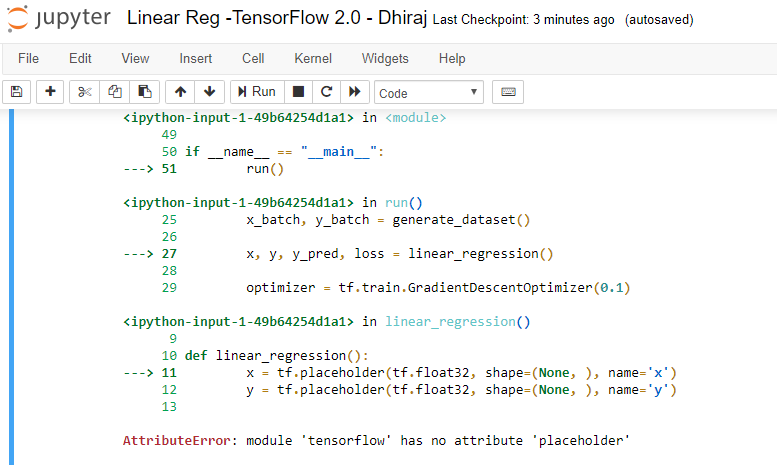If you want to run the existing code (written in version 1.x) with version 2.0, you have two options:

1. Run your TensorFlow 2.0 installation in version 1.0 mode by using the below two lines of code:
``````import tensorflow.compat.v1 as tf
tf.disable_v2_behavior()
``````
1. Modify your code to to work with version 2.0, as well as use the new and exciting features of version 2.0.
Linear Regression with TensorFlow 2.0

In this article, we’re going to use TensorFlow 2.0-compatible code to train a linear regression model.

Linear regression is an algorithm that finds a linear relationship between a dependent variable and one or more independent variables. The dependent variable is also called a label and independent variables are called features.

We’ll start by importing the necessary libraries. Let’s import three, namely `numpy`, `tensorflow`, and `matplotlib`, as shown below:

``````import numpy as np
import tensorflow as tf
import matplotlib.pyplot as plt
``````

Before coding further, let’s make sure we’ve got the current version of TensorFlow ready to go.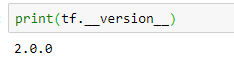Our next step is to create synthetic data for the model, as shown below. Assuming the equation of a line as y = mx + c , note that we’ve taken the slope of the line m as 2 and constant value c as 0.9. There is some error data we’ve introduced using `np.random`, as we don’t want the model to overfit as a straight line — this is because we want the model to work on unseen data.

``````# actual weight = 2 and actual bias = 0.9
x = np.linspace(0, 3, 120)
y = 2 * x + 0.9 + np.random.randn(*x.shape) * 0.3
``````

Let’s plot the data to see if it has linear pattern. We’re using Matplotlib for plotting. The data points below clearly show a pattern we’re looking for. Noticed that the data isn’t a perfectly straight line.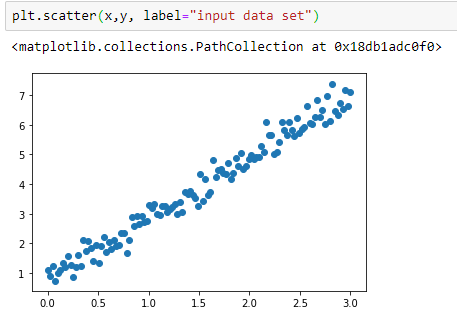After visualizing our data, let’s create a class called `Linear Model` that has two methods: init and call. Init initializes the weight and bias randomly, and call returns the values, as per the straight line equation y = mx + c

``````class LinearModel:
def __call__(self, x):
return self.Weight * x + self.Bias

def __init__(self):
self.Weight = tf.Variable(11.0)
self.Bias = tf.Variable(12.0)
``````

Now let’s define the loss and train functions for the model. The train function takes four parameters: `linear_model` (model instance) , `x` (independent variable) , `y` (dependent variable), and `lr` (learning rate).

The loss function takes two parameters: `y` (actual value of dependent variable) and `pred` (predicted value of dependent variable).

Note that we’re using the `tf.square` function to get the square of the difference of `y` and the predicted value, and then we’re using the . `tf.reduce_mean` method to calculate the square root of the mean.

Note that the `tf.GradientTape` method is used for automatic differentiation, computing the gradient of a computation with respect to its input variables.
Hence, all operations executed inside the context of a `tf.GradientTape` are recorded.

``````def loss(y, pred):
return tf.reduce_mean(tf.square(y - pred))

def train(linear_model, x, y, lr=0.12):
current_loss = loss(y, linear_model(x))

lr_weight, lr_bias = t.gradient(current_loss, [linear_model.Weight, linear_model.Bias])
linear_model.Weight.assign_sub(lr * lr_weight)
linear_model.Bias.assign_sub(lr * lr_bias)
``````

Here we’re defining the number of epochs as 80 and using a for loop to train the model. Note that we’re printing the epoch count and loss for each epoch using that same for loop. We’ve used 0.12 for learning rate, and we’re calculating the loss in each epoch by calling our loss function inside the for loop as shown below.

``````linear_model = LinearModel()
Weights, Biases = [], []
epochs = 80
for epoch_count in range(epochs):
Weights.append(linear_model.Weight.numpy())
Biases.append(linear_model.Bias.numpy())
real_loss = loss(y, linear_model(x))
train(linear_model, x, y, lr=0.12)
print(f"Epoch count {epoch_count}: Loss value: {real_loss.numpy()}")
``````

Below is the output during model training. This shows how our loss value is decreasing as the epoch count is increasing. Note that, initially, the loss was very high as we initialized the model with random values for weight and bias. Once the model starts learning, the loss starts decreasing.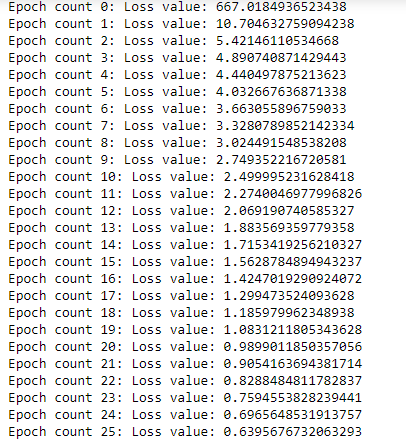And finally, we’d like to know the weight and bias values as well as RMSE for the model, which is shown below.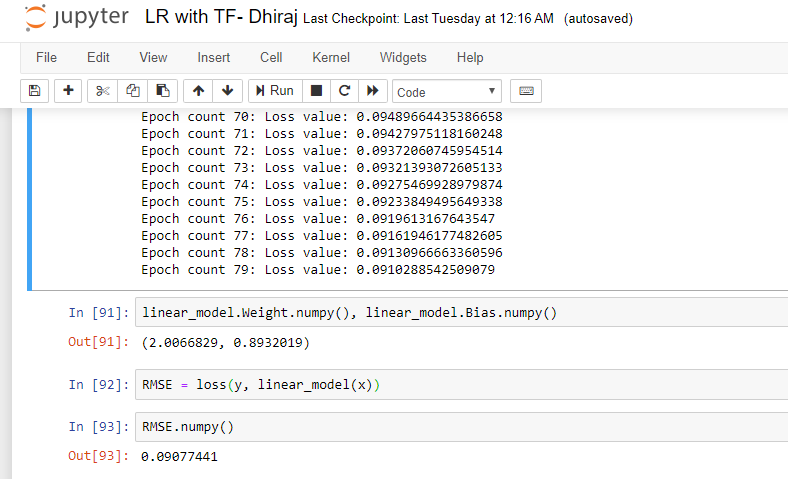End notes

I hope you enjoyed creating and evaluating a linear regression model with TensorFlow 2.0.

You can find the complete code here.

Happy Machine Learning :)

# Introducing TensorFlow DatasetsIntroducing TensorFlow Datasets

Public datasets fuel the machine learning research rocket (h/t Andrew Ng), but it’s still too difficult to simply get those datasets into your machine learning pipeline. Every researcher goes through the pain of writing one-off scripts to download and prepare every dataset they work with, which all have different source formats and complexities. Not anymore.

Today, we’re pleased to introduce TensorFlow Datasets (GitHub) which exposes public research datasets as `[tf.data.Datasets]([https://www.tensorflow.org/api_docs/python/tf/data/Dataset)](https://www.tensorflow.org/api_docs/python/tf/data/Dataset) "https://www.tensorflow.org/api_docs/python/tf/data/Dataset)")` and as NumPy arrays. It does all the grungy work of fetching the source data and preparing it into a common format on disk, and it uses the `[tf.data API]([https://www.tensorflow.org/guide/datasets)](https://www.tensorflow.org/guide/datasets) "https://www.tensorflow.org/guide/datasets)")` to build high-performance input pipelines, which are TensorFlow 2.0-ready and can be used with `tf.keras` models. We’re launching with 29 popular research datasets such as MNIST, Street View House Numbers, the 1 Billion Word Language Model Benchmark, and the Large Movie Reviews Dataset, and will add more in the months to come; we hope that you join in and add a dataset yourself.

### tl;dr

``````# Install: pip install tensorflow-datasets
import tensorflow_datasets as tfds
mnist_train, mnist_test = mnist_data["train"], mnist_data["test"]
assert isinstance(mnist_train, tf.data.Dataset)

``````

Try `tfds` out in a Colab notebook.

### `[tfds.load]([https://www.tensorflow.org/datasets/api_docs/python/tfds/load)](https://www.tensorflow.org/datasets/api_docs/python/tfds/load) "https://www.tensorflow.org/datasets/api_docs/python/tfds/load)")` and `[DatasetBuilder]([https://www.tensorflow.org/datasets/api_docs/python/tfds/core/DatasetBuilder)](https://www.tensorflow.org/datasets/api_docs/python/tfds/core/DatasetBuilder) "https://www.tensorflow.org/datasets/api_docs/python/tfds/core/DatasetBuilder)")`

Every dataset is exposed as a DatasetBuilder, which knows:

• Where to download the data from and how to extract it and write it to a standard format (`[DatasetBuilder.download_and_prepare]([https://www.tensorflow.org/datasets/api_docs/python/tfds/core/DatasetBuilder#download_and_prepare)](https://www.tensorflow.org/datasets/api_docs/python/tfds/core/DatasetBuilder#download_and_prepare) "https://www.tensorflow.org/datasets/api_docs/python/tfds/core/DatasetBuilder#download_and_prepare)")`).
• How to load it from disk (`[DatasetBuilder.as_dataset]([https://www.tensorflow.org/datasets/api_docs/python/tfds/core/DatasetBuilder#as_dataset)](https://www.tensorflow.org/datasets/api_docs/python/tfds/core/DatasetBuilder#as_dataset) "https://www.tensorflow.org/datasets/api_docs/python/tfds/core/DatasetBuilder#as_dataset)")`).
• And all the information about the dataset, like the names, types, and shapes of all the features, the number of records in each split, the source URLs, citation for the dataset or associated paper, etc. (`[DatasetBuilder.info]([https://www.tensorflow.org/datasets/api_docs/python/tfds/core/DatasetBuilder#info)](https://www.tensorflow.org/datasets/api_docs/python/tfds/core/DatasetBuilder#info) "https://www.tensorflow.org/datasets/api_docs/python/tfds/core/DatasetBuilder#info)")`).

You can directly instantiate any of the DatasetBuilders or fetch them by string with `[tfds.builder]([https://www.tensorflow.org/datasets/api_docs/python/tfds/builder)](https://www.tensorflow.org/datasets/api_docs/python/tfds/builder) "https://www.tensorflow.org/datasets/api_docs/python/tfds/builder)")`:

``````import tensorflow_datasets as tfds

# Fetch the dataset directly
mnist = tfds.image.MNIST()
# or by string name
mnist = tfds.builder('mnist')

# Describe the dataset with DatasetInfo
assert mnist.info.features['image'].shape == (28, 28, 1)
assert mnist.info.features['label'].num_classes == 10
assert mnist.info.splits['train'].num_examples == 60000

# Load data from disk as tf.data.Datasets
datasets = mnist.as_dataset()
train_dataset, test_dataset = datasets['train'], datasets['test']
assert isinstance(train_dataset, tf.data.Dataset)

# And convert the Dataset to NumPy arrays if you'd like
for example in tfds.as_numpy(train_dataset):
image, label = example['image'], example['label']
assert isinstance(image, np.array)

``````

`as_dataset()` accepts a `batch_size` argument which will give you batches of examples instead of one example at a time. For small datasets that fit in memory, you can pass `batch_size=-1` to get the entire dataset at once as a `tf.Tensor`. All `tf.data.Datasets` can easily be converted to iterables of NumPy arrays using `[tfds.as_numpy()]([https://www.tensorflow.org/datasets/api_docs/python/tfds/as_numpy)](https://www.tensorflow.org/datasets/api_docs/python/tfds/as_numpy) "https://www.tensorflow.org/datasets/api_docs/python/tfds/as_numpy)")`.

As a convenience, you can do all the above with `[tfds.load]([https://www.tensorflow.org/datasets/api_docs/python/tfds/load)](https://www.tensorflow.org/datasets/api_docs/python/tfds/load) "https://www.tensorflow.org/datasets/api_docs/python/tfds/load)")`, which fetches the DatasetBuilder by name, calls `download_and_prepare()`, and calls `as_dataset()`.

``````import tensorflow_datasets as tfds

train_dataset, test_dataset = datasets["train"], datasets["test"]
assert isinstance(train_dataset, tf.data.Dataset)

``````

You can also easily get the `[DatasetInfo]([https://www.tensorflow.org/datasets/api_docs/python/tfds/core/DatasetInfo)](https://www.tensorflow.org/datasets/api_docs/python/tfds/core/DatasetInfo) "https://www.tensorflow.org/datasets/api_docs/python/tfds/core/DatasetInfo)")` object from `tfds.load` by passing `with_info=True`. See the API documentation for all the options.

### Dataset Versioning

Every dataset is versioned (`builder.info.version`) so that you can rest assured that the data doesn’t change underneath you and that results are reproducible. For now, we guarantee that if the data changes, the version will be incremented.

Note that while we do guarantee the data values and splits are identical given the same version, we do not currently guarantee the ordering of records for the same version.

### Dataset Configuration

Datasets with different variants are configured with named BuilderConfigs. For example, the Large Movie Review Dataset (`[tfds.text.IMDBReviews]([https://www.tensorflow.org/datasets/datasets#imdb_reviews)](https://www.tensorflow.org/datasets/datasets#imdb_reviews) "https://www.tensorflow.org/datasets/datasets#imdb_reviews)")`) could have different encodings for the input text (for example, plain text, or a character encoding, or a subword encoding). The built-in configurations are listed with the dataset documentation and can be addressed by string, or you can pass in your own configuration.

``````# See the built-in configs
configs = tfds.text.IMDBReviews.builder_configs
assert "bytes" in configs

# Address a built-in config with tfds.builder
imdb = tfds.builder("imdb_reviews/bytes")
# or when constructing the builder directly
imdb = tfds.text.IMDBReviews(config="bytes")
# or use your own custom configuration
my_config = tfds.text.IMDBReviewsConfig(
name="my_config",
version="1.0.0",
text_encoder_config=tfds.features.text.TextEncoderConfig(encoder=my_encoder),
)
imdb = tfds.text.IMDBReviews(config=my_config)

``````

See the section on dataset configuration in our documentation on adding a dataset.

### Text Datasets and Vocabularies

Text datasets can be often be painful to work with because of different encodings and vocabulary files. `tensorflow-datasets` makes it much easier. It’s shipping with many text tasks and includes three kinds of TextEncoders, all of which support Unicode:

• Where to download the data from and how to extract it and write it to a standard format (`[DatasetBuilder.download_and_prepare]([https://www.tensorflow.org/datasets/api_docs/python/tfds/core/DatasetBuilder#download_and_prepare)](https://www.tensorflow.org/datasets/api_docs/python/tfds/core/DatasetBuilder#download_and_prepare) "https://www.tensorflow.org/datasets/api_docs/python/tfds/core/DatasetBuilder#download_and_prepare)")`).
• How to load it from disk (`[DatasetBuilder.as_dataset]([https://www.tensorflow.org/datasets/api_docs/python/tfds/core/DatasetBuilder#as_dataset)](https://www.tensorflow.org/datasets/api_docs/python/tfds/core/DatasetBuilder#as_dataset) "https://www.tensorflow.org/datasets/api_docs/python/tfds/core/DatasetBuilder#as_dataset)")`).
• And all the information about the dataset, like the names, types, and shapes of all the features, the number of records in each split, the source URLs, citation for the dataset or associated paper, etc. (`[DatasetBuilder.info]([https://www.tensorflow.org/datasets/api_docs/python/tfds/core/DatasetBuilder#info)](https://www.tensorflow.org/datasets/api_docs/python/tfds/core/DatasetBuilder#info) "https://www.tensorflow.org/datasets/api_docs/python/tfds/core/DatasetBuilder#info)")`).

The encoders, along with their vocabulary sizes, can be accessed through `DatasetInfo`:

``````imdb = tfds.builder("imdb_reviews/subwords8k")

# Get the TextEncoder from DatasetInfo
encoder = imdb.info.features["text"].encoder
assert isinstance(encoder, tfds.features.text.SubwordTextEncoder)

# Encode, decode
ids = encoder.encode("Hello world")
assert encoder.decode(ids) == "Hello world"

# Get the vocabulary size
vocab_size = encoder.vocab_size

``````

Both TensorFlow and TensorFlow Datasets will be working to improve text support even further in the future.

### Getting started

Our documentation site is the best place to start using `tensorflow-datasets`. Here are some additional pointers for getting started:

• Where to download the data from and how to extract it and write it to a standard format (`[DatasetBuilder.download_and_prepare]([https://www.tensorflow.org/datasets/api_docs/python/tfds/core/DatasetBuilder#download_and_prepare)](https://www.tensorflow.org/datasets/api_docs/python/tfds/core/DatasetBuilder#download_and_prepare) "https://www.tensorflow.org/datasets/api_docs/python/tfds/core/DatasetBuilder#download_and_prepare)")`).
• How to load it from disk (`[DatasetBuilder.as_dataset]([https://www.tensorflow.org/datasets/api_docs/python/tfds/core/DatasetBuilder#as_dataset)](https://www.tensorflow.org/datasets/api_docs/python/tfds/core/DatasetBuilder#as_dataset) "https://www.tensorflow.org/datasets/api_docs/python/tfds/core/DatasetBuilder#as_dataset)")`).
• And all the information about the dataset, like the names, types, and shapes of all the features, the number of records in each split, the source URLs, citation for the dataset or associated paper, etc. (`[DatasetBuilder.info]([https://www.tensorflow.org/datasets/api_docs/python/tfds/core/DatasetBuilder#info)](https://www.tensorflow.org/datasets/api_docs/python/tfds/core/DatasetBuilder#info) "https://www.tensorflow.org/datasets/api_docs/python/tfds/core/DatasetBuilder#info)")`).

We expect to be adding datasets in the coming months, and we hope that the community will join in. Open a GitHub Issue to request a dataset, vote on which datasets should be added next, discuss implementation, or ask for help. And Pull Requests very welcome! Add a popular dataset to contribute to the community, or if you have your own data, contribute it to TFDS to make your data famous!

Now that data is easy, happy modeling!

### Acknowledgements

We’d like to thank Stefan Webb of Oxford for allowing us to use the `tensorflow-datasets` PyPI name. Thanks Stefan!

We’d also like to thank Lukasz Kaiser and the Tensor2Tensor project for inspiring and guiding tensorflow/datasets. Thanks Lukasz! T2T will be migrating to tensorflow/datasets soon.

# Introduction to the Python Deep Learning Library TensorFlow for BeginnersIn this tutorial, we are going to be covering some basics on what TensorFlow is, and how to begin using it.

TensorFlow is a Python library for fast numerical computing created and released by Google.

It is a foundation library that can be used to create Deep Learning models directly or by using wrapper libraries that simplify the process built on top of TensorFlow.

In this post you will discover the TensorFlow library for Deep Learning.

Discover how to develop deep learning models for a range of predictive modeling problems with just a few lines of code in my new book, with 18 step-by-step tutorials and 9 projects.

What is TensorFlow?

TensorFlow is an open source library for fast numerical computing.

It was created and is maintained by Google and released under the Apache 2.0 open source license. The API is nominally for the Python programming language, although there is access to the underlying C++ API.

Unlike other numerical libraries intended for use in Deep Learning like Theano, TensorFlow was designed for use both in research and development and in production systems, not least RankBrain in Google search and the fun DeepDream project.

It can run on single CPU systems, GPUs as well as mobile devices and large scale distributed systems of hundreds of machines.

You may also like: Deep Learning Using TensorFlow

How to Install TensorFlow

Installation of TensorFlow is straightforward if you already have a Python SciPy environment.

TensorFlow works with Python 2.7 and Python 3.3+. You can follow the Download and Setup instructions on the TensorFlow website. Installation is probably simplest via PyPI and specific instructions of the pip command to use for your Linux or Mac OS X platform are on the Download and Setup webpage.

There are also virtualenv and docker images that you can use if you prefer.

To make use of the GPU, only Linux is supported and it requires the Cuda Toolkit.

Computation is described in terms of data flow and operations in the structure of a directed graph.

• Nodes: Nodes perform computation and have zero or more inputs and outputs. Data that moves between nodes are known as tensors, which are multi-dimensional arrays of real values.
• Edges: The graph defines the flow of data, branching, looping and updates to state. Special edges can be used to synchronize behavior within the graph, for example waiting for computation on a number of inputs to complete.
• Operation: An operation is a named abstract computation which can take input attributes and produce output attributes. For example, you could define an add or multiply operation.
Computation with TensorFlow

This first example is a modified version of the example on the TensorFlow website. It shows how you can create a session, define constants and perform computation with those constants using the session.

``````import tensorflow as tf
sess = tf.Session()
a = tf.constant(10)
b = tf.constant(32)
print(sess.run(a+b))
``````

Running this example displays:

``````42
``````
Linear Regression with TensorFlow

This next example comes from the introduction on the TensorFlow tutorial.

This examples shows how you can define variables (e.g. W and b) as well as variables that are the result of computation (y).

We get some sense of TensorFlow separates the definition and declaration of the computation from the execution in the session and the calls to run.

``````import tensorflow as tf
import numpy as np

# Create 100 phony x, y data points in NumPy, y = x * 0.1 + 0.3
x_data = np.random.rand(100).astype(np.float32)
y_data = x_data * 0.1 + 0.3

# Try to find values for W and b that compute y_data = W * x_data + b
# (We know that W should be 0.1 and b 0.3, but Tensorflow will
# figure that out for us.)
W = tf.Variable(tf.random_uniform(, -1.0, 1.0))
b = tf.Variable(tf.zeros())
y = W * x_data + b

# Minimize the mean squared errors.
loss = tf.reduce_mean(tf.square(y - y_data))
train = optimizer.minimize(loss)

# Before starting, initialize the variables.  We will 'run' this first.
init = tf.initialize_all_variables()

# Launch the graph.
sess = tf.Session()
sess.run(init)

# Fit the line.
for step in xrange(201):
sess.run(train)
if step % 20 == 0:
print(step, sess.run(W), sess.run(b))

# Learns best fit is W: [0.1], b: [0.3]
``````

Running this example prints the following output:

``````(0, array([ 0.2629351], dtype=float32), array([ 0.28697217], dtype=float32))
(20, array([ 0.13929555], dtype=float32), array([ 0.27992988], dtype=float32))
(40, array([ 0.11148042], dtype=float32), array([ 0.2941364], dtype=float32))
(60, array([ 0.10335406], dtype=float32), array([ 0.29828694], dtype=float32))
(80, array([ 0.1009799], dtype=float32), array([ 0.29949954], dtype=float32))
(100, array([ 0.10028629], dtype=float32), array([ 0.2998538], dtype=float32))
(120, array([ 0.10008363], dtype=float32), array([ 0.29995731], dtype=float32))
(140, array([ 0.10002445], dtype=float32), array([ 0.29998752], dtype=float32))
(160, array([ 0.10000713], dtype=float32), array([ 0.29999638], dtype=float32))
(180, array([ 0.10000207], dtype=float32), array([ 0.29999897], dtype=float32))
(200, array([ 0.1000006], dtype=float32), array([ 0.29999971], dtype=float32))
``````

More Deep Learning Models

Your TensorFlow installation comes with a number of Deep Learning models that you can use and experiment with directly.

Firstly, you need to find out where TensorFlow was installed on your system. For example, you can use the following Python script:

``````python -c 'import os; import inspect; import tensorflow; print(os.path.dirname(inspect.getfile(tensorflow)))'
``````

For example, this could be:

``````/usr/lib/python2.7/site-packages/tensorflow
``````

Change to this directory and take note of the models subdirectory. Included are a number of deep learning models with tutorial-like comments, such as:

• Multi-threaded word2vec mini-batched skip-gram model.
• Multi-threaded word2vec unbatched skip-gram model.
• CNN for the CIFAR-10 network.
• Simple, end-to-end, LeNet-5-like convolutional MNIST model example.
• Sequence-to-sequence model with an attention mechanism.

Also check the examples directory as it contains an example using the MNIST dataset.

There is also an excellent list of tutorials on the main TensorFlow website. They show how to use different network types, different datasets and how to use the framework in various different ways.

Summary

In this post you discovered the TensorFlow Python library for deep learning.

You learned that it is a library for fast numerical computation, specifically designed for the types of operations that are required in the development and evaluation of large deep learning models.

Deploying a Keras Deep Learning Model as a Web Application in Python

Deep Learning With TensorFlow 2.0

# Creating Chatbots Tutorial Using TensorFlow 2.0In this tutorial, you will learn how to build a transformer chatbot using TensorFlow 2.0

The use of artificial neural networks to create **chatbots **is increasingly popular nowadays, however, teaching a computer to have natural conversations is very difficult and often requires large and complicated language models.

With all the changes and improvements made in **TensorFlow 2.0 **we can build complicated models with ease. In this post, we will demonstrate how to build a Transformer chatbot. All of the code used in this post is available in this colab notebook, which will run end to end (including installing TensorFlow 2.0).

This article assumes some knowledge of text generation, attention and transformer. In this tutorial we are going to focus on:

• Preprocessing the Cornell Movie-Dialogs Corpus using **TensorFlow **Datasets and creating an input pipeline using `tf.data`
• Implementing MultiHeadAttention with Model subclassing
• Implementing a Transformer with Functional API
``````input: where have you been ?
output: i m not talking about that .
input: i am not crazy , my mother had me tested .
output: i m not sure . i m not hungry .
input: i m not sure . i m not hungry .
output: you re a liar .
input: you re a liar .
output: i m not going to be a man . i m gonna need to go to school .

``````

Sample conversations of a Transformer chatbot trained on Movie-Dialogs Corpus.

Transformer

Transformer, proposed in the paper Attention is All You Need, is a neural network architecture solely based on self-attention mechanism and is very parallelizable.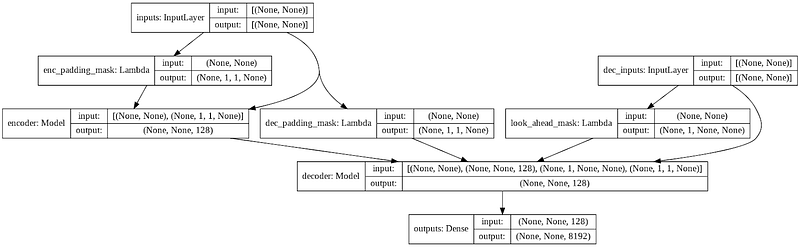tf.keras model plot of our Transformer

A Transformer model handles variable-sized input using stacks of self-attention layers instead of RNNs or CNNs. This general architecture has a number of advantages:

• It makes no assumptions about the temporal/spatial relationships across the data. This is ideal for processing a set of objects.
• Layer outputs can be calculated in parallel, instead of a series like an RNN.
• Distant items can affect each other’s output without passing through many recurrent steps, or convolution layers.
• It can learn long-range dependencies.

• For a time-series, the output for a time-step is calculated from the entire history instead of only the inputs and current hidden-state. This may be less efficient.
• If the input does have a temporal/spatial relationship, like text, some positional encoding must be added or the model will effectively see a bag of words.

If you are interested in knowing more about Transformer, check out The Annotated Transformer and Illustrated Transformer.

Dataset

We are using the Cornell Movie-Dialogs Corpus as our dataset, which contains more than 220k conversational exchanges between more than 10k pairs of movie characters.

“+++\$+++” is being used as a field separator in all the files within the corpus dataset.

`movie_conversations.txt` has the following format: ID of the first character, ID of the second character, ID of the movie that this conversation occurred, and a list of line IDs. The character and movie information can be found in `movie_characters_metadata.txt` and `movie_titles_metadata.txt` respectively.

``````u0 +++u0 +++\$+++ u2 +++\$+++ m0 +++\$+++ [‘L194’, ‘L195’, ‘L196’, ‘L197’]
u0 +++\$+++ u2 +++\$+++ m0 +++\$+++ [‘L198’, ‘L199’]
u0 +++\$+++ u2 +++\$+++ m0 +++\$+++ [‘L200’, ‘L201’, ‘L202’, ‘L203’]
u0 +++\$+++ u2 +++\$+++ m0 +++\$+++ [‘L204’, ‘L205’, ‘L206’]
u0 +++\$+++ u2 +++\$+++ m0 +++\$+++ [‘L207’, ‘L208’]
++ u2 +++u0 +++\$+++ u2 +++\$+++ m0 +++\$+++ [‘L194’, ‘L195’, ‘L196’, ‘L197’]
u0 +++\$+++ u2 +++\$+++ m0 +++\$+++ [‘L198’, ‘L199’]
u0 +++\$+++ u2 +++\$+++ m0 +++\$+++ [‘L200’, ‘L201’, ‘L202’, ‘L203’]
u0 +++\$+++ u2 +++\$+++ m0 +++\$+++ [‘L204’, ‘L205’, ‘L206’]
u0 +++\$+++ u2 +++\$+++ m0 +++\$+++ [‘L207’, ‘L208’]
++ m0 +++u0 +++\$+++ u2 +++\$+++ m0 +++\$+++ [‘L194’, ‘L195’, ‘L196’, ‘L197’]
u0 +++\$+++ u2 +++\$+++ m0 +++\$+++ [‘L198’, ‘L199’]
u0 +++\$+++ u2 +++\$+++ m0 +++\$+++ [‘L200’, ‘L201’, ‘L202’, ‘L203’]
u0 +++\$+++ u2 +++\$+++ m0 +++\$+++ [‘L204’, ‘L205’, ‘L206’]
u0 +++\$+++ u2 +++\$+++ m0 +++\$+++ [‘L207’, ‘L208’]
++ [‘L194’, ‘L195’, ‘L196’, ‘L197’]
u0 +++u0 +++\$+++ u2 +++\$+++ m0 +++\$+++ [‘L194’, ‘L195’, ‘L196’, ‘L197’]
u0 +++\$+++ u2 +++\$+++ m0 +++\$+++ [‘L198’, ‘L199’]
u0 +++\$+++ u2 +++\$+++ m0 +++\$+++ [‘L200’, ‘L201’, ‘L202’, ‘L203’]
u0 +++\$+++ u2 +++\$+++ m0 +++\$+++ [‘L204’, ‘L205’, ‘L206’]
u0 +++\$+++ u2 +++\$+++ m0 +++\$+++ [‘L207’, ‘L208’]
++ u2 +++u0 +++\$+++ u2 +++\$+++ m0 +++\$+++ [‘L194’, ‘L195’, ‘L196’, ‘L197’]
u0 +++\$+++ u2 +++\$+++ m0 +++\$+++ [‘L198’, ‘L199’]
u0 +++\$+++ u2 +++\$+++ m0 +++\$+++ [‘L200’, ‘L201’, ‘L202’, ‘L203’]
u0 +++\$+++ u2 +++\$+++ m0 +++\$+++ [‘L204’, ‘L205’, ‘L206’]
u0 +++\$+++ u2 +++\$+++ m0 +++\$+++ [‘L207’, ‘L208’]
++ m0 +++u0 +++\$+++ u2 +++\$+++ m0 +++\$+++ [‘L194’, ‘L195’, ‘L196’, ‘L197’]
u0 +++\$+++ u2 +++\$+++ m0 +++\$+++ [‘L198’, ‘L199’]
u0 +++\$+++ u2 +++\$+++ m0 +++\$+++ [‘L200’, ‘L201’, ‘L202’, ‘L203’]
u0 +++\$+++ u2 +++\$+++ m0 +++\$+++ [‘L204’, ‘L205’, ‘L206’]
u0 +++\$+++ u2 +++\$+++ m0 +++\$+++ [‘L207’, ‘L208’]
++ [‘L198’, ‘L199’]
u0 +++u0 +++\$+++ u2 +++\$+++ m0 +++\$+++ [‘L194’, ‘L195’, ‘L196’, ‘L197’]
u0 +++\$+++ u2 +++\$+++ m0 +++\$+++ [‘L198’, ‘L199’]
u0 +++\$+++ u2 +++\$+++ m0 +++\$+++ [‘L200’, ‘L201’, ‘L202’, ‘L203’]
u0 +++\$+++ u2 +++\$+++ m0 +++\$+++ [‘L204’, ‘L205’, ‘L206’]
u0 +++\$+++ u2 +++\$+++ m0 +++\$+++ [‘L207’, ‘L208’]
++ u2 +++u0 +++\$+++ u2 +++\$+++ m0 +++\$+++ [‘L194’, ‘L195’, ‘L196’, ‘L197’]
u0 +++\$+++ u2 +++\$+++ m0 +++\$+++ [‘L198’, ‘L199’]
u0 +++\$+++ u2 +++\$+++ m0 +++\$+++ [‘L200’, ‘L201’, ‘L202’, ‘L203’]
u0 +++\$+++ u2 +++\$+++ m0 +++\$+++ [‘L204’, ‘L205’, ‘L206’]
u0 +++\$+++ u2 +++\$+++ m0 +++\$+++ [‘L207’, ‘L208’]
++ m0 +++u0 +++\$+++ u2 +++\$+++ m0 +++\$+++ [‘L194’, ‘L195’, ‘L196’, ‘L197’]
u0 +++\$+++ u2 +++\$+++ m0 +++\$+++ [‘L198’, ‘L199’]
u0 +++\$+++ u2 +++\$+++ m0 +++\$+++ [‘L200’, ‘L201’, ‘L202’, ‘L203’]
u0 +++\$+++ u2 +++\$+++ m0 +++\$+++ [‘L204’, ‘L205’, ‘L206’]
u0 +++\$+++ u2 +++\$+++ m0 +++\$+++ [‘L207’, ‘L208’]
++ [‘L200’, ‘L201’, ‘L202’, ‘L203’]
u0 +++u0 +++\$+++ u2 +++\$+++ m0 +++\$+++ [‘L194’, ‘L195’, ‘L196’, ‘L197’]
u0 +++\$+++ u2 +++\$+++ m0 +++\$+++ [‘L198’, ‘L199’]
u0 +++\$+++ u2 +++\$+++ m0 +++\$+++ [‘L200’, ‘L201’, ‘L202’, ‘L203’]
u0 +++\$+++ u2 +++\$+++ m0 +++\$+++ [‘L204’, ‘L205’, ‘L206’]
u0 +++\$+++ u2 +++\$+++ m0 +++\$+++ [‘L207’, ‘L208’]
++ u2 +++u0 +++\$+++ u2 +++\$+++ m0 +++\$+++ [‘L194’, ‘L195’, ‘L196’, ‘L197’]
u0 +++\$+++ u2 +++\$+++ m0 +++\$+++ [‘L198’, ‘L199’]
u0 +++\$+++ u2 +++\$+++ m0 +++\$+++ [‘L200’, ‘L201’, ‘L202’, ‘L203’]
u0 +++\$+++ u2 +++\$+++ m0 +++\$+++ [‘L204’, ‘L205’, ‘L206’]
u0 +++\$+++ u2 +++\$+++ m0 +++\$+++ [‘L207’, ‘L208’]
++ m0 +++u0 +++\$+++ u2 +++\$+++ m0 +++\$+++ [‘L194’, ‘L195’, ‘L196’, ‘L197’]
u0 +++\$+++ u2 +++\$+++ m0 +++\$+++ [‘L198’, ‘L199’]
u0 +++\$+++ u2 +++\$+++ m0 +++\$+++ [‘L200’, ‘L201’, ‘L202’, ‘L203’]
u0 +++\$+++ u2 +++\$+++ m0 +++\$+++ [‘L204’, ‘L205’, ‘L206’]
u0 +++\$+++ u2 +++\$+++ m0 +++\$+++ [‘L207’, ‘L208’]
++ [‘L204’, ‘L205’, ‘L206’]
u0 +++u0 +++\$+++ u2 +++\$+++ m0 +++\$+++ [‘L194’, ‘L195’, ‘L196’, ‘L197’]
u0 +++\$+++ u2 +++\$+++ m0 +++\$+++ [‘L198’, ‘L199’]
u0 +++\$+++ u2 +++\$+++ m0 +++\$+++ [‘L200’, ‘L201’, ‘L202’, ‘L203’]
u0 +++\$+++ u2 +++\$+++ m0 +++\$+++ [‘L204’, ‘L205’, ‘L206’]
u0 +++\$+++ u2 +++\$+++ m0 +++\$+++ [‘L207’, ‘L208’]
++ u2 +++u0 +++\$+++ u2 +++\$+++ m0 +++\$+++ [‘L194’, ‘L195’, ‘L196’, ‘L197’]
u0 +++\$+++ u2 +++\$+++ m0 +++\$+++ [‘L198’, ‘L199’]
u0 +++\$+++ u2 +++\$+++ m0 +++\$+++ [‘L200’, ‘L201’, ‘L202’, ‘L203’]
u0 +++\$+++ u2 +++\$+++ m0 +++\$+++ [‘L204’, ‘L205’, ‘L206’]
u0 +++\$+++ u2 +++\$+++ m0 +++\$+++ [‘L207’, ‘L208’]
++ m0 +++u0 +++\$+++ u2 +++\$+++ m0 +++\$+++ [‘L194’, ‘L195’, ‘L196’, ‘L197’]
u0 +++\$+++ u2 +++\$+++ m0 +++\$+++ [‘L198’, ‘L199’]
u0 +++\$+++ u2 +++\$+++ m0 +++\$+++ [‘L200’, ‘L201’, ‘L202’, ‘L203’]
u0 +++\$+++ u2 +++\$+++ m0 +++\$+++ [‘L204’, ‘L205’, ‘L206’]
u0 +++\$+++ u2 +++\$+++ m0 +++\$+++ [‘L207’, ‘L208’]
++ [‘L207’, ‘L208’]

``````

*Samples of conversations pairs from *`<em>movie_conversations.txt</em>`

`movie_lines.txt` has the following format: ID of the conversation line, ID of the character who uttered this phase, ID of the movie, name of the character and the text of the line.

``````L901 +++L901 +++\$+++ u5 +++\$+++ m0 +++\$+++ KAT +++\$+++ He said everyone was doing it. So I did it.
L900 +++\$+++ u0 +++\$+++ m0 +++\$+++ BIANCA +++\$+++ As in…
L899 +++\$+++ u5 +++\$+++ m0 +++\$+++ KAT +++\$+++ Now I do. Back then, was a different story.
L898 +++\$+++ u0 +++\$+++ m0 +++\$+++ BIANCA +++\$+++ But you hate Joey
L897 +++\$+++ u5 +++\$+++ m0 +++\$+++ KAT +++\$+++ He was, like, a total babe
++ u5 +++L901 +++\$+++ u5 +++\$+++ m0 +++\$+++ KAT +++\$+++ He said everyone was doing it. So I did it.
L900 +++\$+++ u0 +++\$+++ m0 +++\$+++ BIANCA +++\$+++ As in…
L899 +++\$+++ u5 +++\$+++ m0 +++\$+++ KAT +++\$+++ Now I do. Back then, was a different story.
L898 +++\$+++ u0 +++\$+++ m0 +++\$+++ BIANCA +++\$+++ But you hate Joey
L897 +++\$+++ u5 +++\$+++ m0 +++\$+++ KAT +++\$+++ He was, like, a total babe
++ m0 +++L901 +++\$+++ u5 +++\$+++ m0 +++\$+++ KAT +++\$+++ He said everyone was doing it. So I did it.
L900 +++\$+++ u0 +++\$+++ m0 +++\$+++ BIANCA +++\$+++ As in…
L899 +++\$+++ u5 +++\$+++ m0 +++\$+++ KAT +++\$+++ Now I do. Back then, was a different story.
L898 +++\$+++ u0 +++\$+++ m0 +++\$+++ BIANCA +++\$+++ But you hate Joey
L897 +++\$+++ u5 +++\$+++ m0 +++\$+++ KAT +++\$+++ He was, like, a total babe
++ KAT +++L901 +++\$+++ u5 +++\$+++ m0 +++\$+++ KAT +++\$+++ He said everyone was doing it. So I did it.
L900 +++\$+++ u0 +++\$+++ m0 +++\$+++ BIANCA +++\$+++ As in…
L899 +++\$+++ u5 +++\$+++ m0 +++\$+++ KAT +++\$+++ Now I do. Back then, was a different story.
L898 +++\$+++ u0 +++\$+++ m0 +++\$+++ BIANCA +++\$+++ But you hate Joey
L897 +++\$+++ u5 +++\$+++ m0 +++\$+++ KAT +++\$+++ He was, like, a total babe
++ He said everyone was doing it. So I did it.
L900 +++L901 +++\$+++ u5 +++\$+++ m0 +++\$+++ KAT +++\$+++ He said everyone was doing it. So I did it.
L900 +++\$+++ u0 +++\$+++ m0 +++\$+++ BIANCA +++\$+++ As in…
L899 +++\$+++ u5 +++\$+++ m0 +++\$+++ KAT +++\$+++ Now I do. Back then, was a different story.
L898 +++\$+++ u0 +++\$+++ m0 +++\$+++ BIANCA +++\$+++ But you hate Joey
L897 +++\$+++ u5 +++\$+++ m0 +++\$+++ KAT +++\$+++ He was, like, a total babe
++ u0 +++L901 +++\$+++ u5 +++\$+++ m0 +++\$+++ KAT +++\$+++ He said everyone was doing it. So I did it.
L900 +++\$+++ u0 +++\$+++ m0 +++\$+++ BIANCA +++\$+++ As in…
L899 +++\$+++ u5 +++\$+++ m0 +++\$+++ KAT +++\$+++ Now I do. Back then, was a different story.
L898 +++\$+++ u0 +++\$+++ m0 +++\$+++ BIANCA +++\$+++ But you hate Joey
L897 +++\$+++ u5 +++\$+++ m0 +++\$+++ KAT +++\$+++ He was, like, a total babe
++ m0 +++L901 +++\$+++ u5 +++\$+++ m0 +++\$+++ KAT +++\$+++ He said everyone was doing it. So I did it.
L900 +++\$+++ u0 +++\$+++ m0 +++\$+++ BIANCA +++\$+++ As in…
L899 +++\$+++ u5 +++\$+++ m0 +++\$+++ KAT +++\$+++ Now I do. Back then, was a different story.
L898 +++\$+++ u0 +++\$+++ m0 +++\$+++ BIANCA +++\$+++ But you hate Joey
L897 +++\$+++ u5 +++\$+++ m0 +++\$+++ KAT +++\$+++ He was, like, a total babe
++ BIANCA +++L901 +++\$+++ u5 +++\$+++ m0 +++\$+++ KAT +++\$+++ He said everyone was doing it. So I did it.
L900 +++\$+++ u0 +++\$+++ m0 +++\$+++ BIANCA +++\$+++ As in…
L899 +++\$+++ u5 +++\$+++ m0 +++\$+++ KAT +++\$+++ Now I do. Back then, was a different story.
L898 +++\$+++ u0 +++\$+++ m0 +++\$+++ BIANCA +++\$+++ But you hate Joey
L897 +++\$+++ u5 +++\$+++ m0 +++\$+++ KAT +++\$+++ He was, like, a total babe
++ As in…
L899 +++L901 +++\$+++ u5 +++\$+++ m0 +++\$+++ KAT +++\$+++ He said everyone was doing it. So I did it.
L900 +++\$+++ u0 +++\$+++ m0 +++\$+++ BIANCA +++\$+++ As in…
L899 +++\$+++ u5 +++\$+++ m0 +++\$+++ KAT +++\$+++ Now I do. Back then, was a different story.
L898 +++\$+++ u0 +++\$+++ m0 +++\$+++ BIANCA +++\$+++ But you hate Joey
L897 +++\$+++ u5 +++\$+++ m0 +++\$+++ KAT +++\$+++ He was, like, a total babe
++ u5 +++L901 +++\$+++ u5 +++\$+++ m0 +++\$+++ KAT +++\$+++ He said everyone was doing it. So I did it.
L900 +++\$+++ u0 +++\$+++ m0 +++\$+++ BIANCA +++\$+++ As in…
L899 +++\$+++ u5 +++\$+++ m0 +++\$+++ KAT +++\$+++ Now I do. Back then, was a different story.
L898 +++\$+++ u0 +++\$+++ m0 +++\$+++ BIANCA +++\$+++ But you hate Joey
L897 +++\$+++ u5 +++\$+++ m0 +++\$+++ KAT +++\$+++ He was, like, a total babe
++ m0 +++L901 +++\$+++ u5 +++\$+++ m0 +++\$+++ KAT +++\$+++ He said everyone was doing it. So I did it.
L900 +++\$+++ u0 +++\$+++ m0 +++\$+++ BIANCA +++\$+++ As in…
L899 +++\$+++ u5 +++\$+++ m0 +++\$+++ KAT +++\$+++ Now I do. Back then, was a different story.
L898 +++\$+++ u0 +++\$+++ m0 +++\$+++ BIANCA +++\$+++ But you hate Joey
L897 +++\$+++ u5 +++\$+++ m0 +++\$+++ KAT +++\$+++ He was, like, a total babe
++ KAT +++L901 +++\$+++ u5 +++\$+++ m0 +++\$+++ KAT +++\$+++ He said everyone was doing it. So I did it.
L900 +++\$+++ u0 +++\$+++ m0 +++\$+++ BIANCA +++\$+++ As in…
L899 +++\$+++ u5 +++\$+++ m0 +++\$+++ KAT +++\$+++ Now I do. Back then, was a different story.
L898 +++\$+++ u0 +++\$+++ m0 +++\$+++ BIANCA +++\$+++ But you hate Joey
L897 +++\$+++ u5 +++\$+++ m0 +++\$+++ KAT +++\$+++ He was, like, a total babe
++ Now I do. Back then, was a different story.
L898 +++L901 +++\$+++ u5 +++\$+++ m0 +++\$+++ KAT +++\$+++ He said everyone was doing it. So I did it.
L900 +++\$+++ u0 +++\$+++ m0 +++\$+++ BIANCA +++\$+++ As in…
L899 +++\$+++ u5 +++\$+++ m0 +++\$+++ KAT +++\$+++ Now I do. Back then, was a different story.
L898 +++\$+++ u0 +++\$+++ m0 +++\$+++ BIANCA +++\$+++ But you hate Joey
L897 +++\$+++ u5 +++\$+++ m0 +++\$+++ KAT +++\$+++ He was, like, a total babe
++ u0 +++L901 +++\$+++ u5 +++\$+++ m0 +++\$+++ KAT +++\$+++ He said everyone was doing it. So I did it.
L900 +++\$+++ u0 +++\$+++ m0 +++\$+++ BIANCA +++\$+++ As in…
L899 +++\$+++ u5 +++\$+++ m0 +++\$+++ KAT +++\$+++ Now I do. Back then, was a different story.
L898 +++\$+++ u0 +++\$+++ m0 +++\$+++ BIANCA +++\$+++ But you hate Joey
L897 +++\$+++ u5 +++\$+++ m0 +++\$+++ KAT +++\$+++ He was, like, a total babe
++ m0 +++L901 +++\$+++ u5 +++\$+++ m0 +++\$+++ KAT +++\$+++ He said everyone was doing it. So I did it.
L900 +++\$+++ u0 +++\$+++ m0 +++\$+++ BIANCA +++\$+++ As in…
L899 +++\$+++ u5 +++\$+++ m0 +++\$+++ KAT +++\$+++ Now I do. Back then, was a different story.
L898 +++\$+++ u0 +++\$+++ m0 +++\$+++ BIANCA +++\$+++ But you hate Joey
L897 +++\$+++ u5 +++\$+++ m0 +++\$+++ KAT +++\$+++ He was, like, a total babe
++ BIANCA +++L901 +++\$+++ u5 +++\$+++ m0 +++\$+++ KAT +++\$+++ He said everyone was doing it. So I did it.
L900 +++\$+++ u0 +++\$+++ m0 +++\$+++ BIANCA +++\$+++ As in…
L899 +++\$+++ u5 +++\$+++ m0 +++\$+++ KAT +++\$+++ Now I do. Back then, was a different story.
L898 +++\$+++ u0 +++\$+++ m0 +++\$+++ BIANCA +++\$+++ But you hate Joey
L897 +++\$+++ u5 +++\$+++ m0 +++\$+++ KAT +++\$+++ He was, like, a total babe
++ But you hate Joey
L897 +++L901 +++\$+++ u5 +++\$+++ m0 +++\$+++ KAT +++\$+++ He said everyone was doing it. So I did it.
L900 +++\$+++ u0 +++\$+++ m0 +++\$+++ BIANCA +++\$+++ As in…
L899 +++\$+++ u5 +++\$+++ m0 +++\$+++ KAT +++\$+++ Now I do. Back then, was a different story.
L898 +++\$+++ u0 +++\$+++ m0 +++\$+++ BIANCA +++\$+++ But you hate Joey
L897 +++\$+++ u5 +++\$+++ m0 +++\$+++ KAT +++\$+++ He was, like, a total babe
++ u5 +++L901 +++\$+++ u5 +++\$+++ m0 +++\$+++ KAT +++\$+++ He said everyone was doing it. So I did it.
L900 +++\$+++ u0 +++\$+++ m0 +++\$+++ BIANCA +++\$+++ As in…
L899 +++\$+++ u5 +++\$+++ m0 +++\$+++ KAT +++\$+++ Now I do. Back then, was a different story.
L898 +++\$+++ u0 +++\$+++ m0 +++\$+++ BIANCA +++\$+++ But you hate Joey
L897 +++\$+++ u5 +++\$+++ m0 +++\$+++ KAT +++\$+++ He was, like, a total babe
++ m0 +++L901 +++\$+++ u5 +++\$+++ m0 +++\$+++ KAT +++\$+++ He said everyone was doing it. So I did it.
L900 +++\$+++ u0 +++\$+++ m0 +++\$+++ BIANCA +++\$+++ As in…
L899 +++\$+++ u5 +++\$+++ m0 +++\$+++ KAT +++\$+++ Now I do. Back then, was a different story.
L898 +++\$+++ u0 +++\$+++ m0 +++\$+++ BIANCA +++\$+++ But you hate Joey
L897 +++\$+++ u5 +++\$+++ m0 +++\$+++ KAT +++\$+++ He was, like, a total babe
++ KAT +++L901 +++\$+++ u5 +++\$+++ m0 +++\$+++ KAT +++\$+++ He said everyone was doing it. So I did it.
L900 +++\$+++ u0 +++\$+++ m0 +++\$+++ BIANCA +++\$+++ As in…
L899 +++\$+++ u5 +++\$+++ m0 +++\$+++ KAT +++\$+++ Now I do. Back then, was a different story.
L898 +++\$+++ u0 +++\$+++ m0 +++\$+++ BIANCA +++\$+++ But you hate Joey
L897 +++\$+++ u5 +++\$+++ m0 +++\$+++ KAT +++\$+++ He was, like, a total babe
++ He was, like, a total babe

``````

*Samples of conversation text from *`<em>movie_lines.txt</em>`

We are going to build the input pipeline with the following steps:

• Extract a list of conversation pairs from `move_conversations.txt` and `movie_lines.txt`
• Preprocess each sentence by removing special characters in each sentence.
• Build tokenizer (map text to ID and ID to text) with TensorFlow Datasets SubwordTextEncoder.
• Tokenize each sentence and add `START_TOKEN` and `END_TOKEN` to indicate the start and end of each sentence.
• Filter out sentences that contain more than `MAX_LENGTH `tokens.
• Pad tokenized sentences to `MAX_LENGTH`
• Build `tf.data.Dataset` with the tokenized sentences

Notice that Transformer is an autoregressive model, it makes predictions one part at a time and uses its output so far to decide what to do next. During training this example uses teacher-forcing. Teacher forcing is passing the true output to the next time step regardless of what the model predicts at the current time step.

The full preprocessing code can be found at the Prepare Dataset section of the colab notebook.

``````i really , really , really wanna go , but i can t . not unless my sister goes .
i m workin on it . but she doesn t seem to be goin for him .

``````

Sample preprocessed conversation pair

Attention

Like many sequence-to-sequence models, Transformer also consist of encoder and decoder. However, instead of recurrent or convolution layers, Transformer uses multi-head attention layers, which consist of multiple scaled dot-product attention.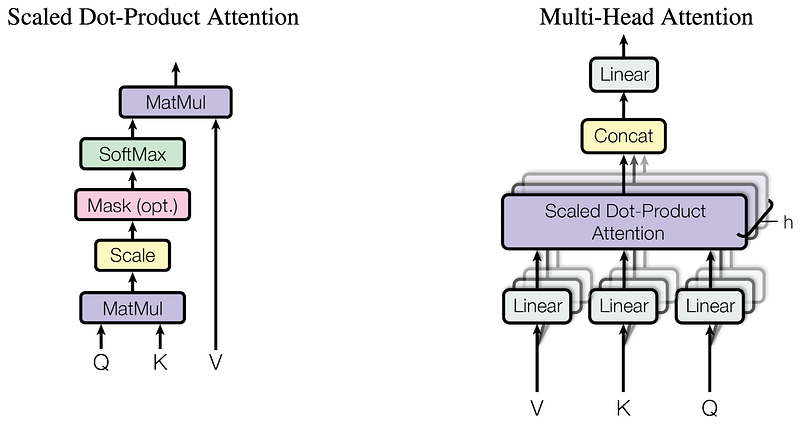Attention architecture diagrams from Attention is All You Need

Scaled dot product attention

The scaled dot-product attention function takes three inputs: Q (`query`), K (`key`), V (`value`). The equation used to calculate the attention weights is:As the softmax normalization being applied on the `key`, its values decide the amount of importance given to the `query`. The output represents the multiplication of the attention weights and `value`. This ensures that the words we want to focus on are kept as is and the irrelevant words are flushed out.

``````
matmul_qk = tf.matmul(query, key, transpose_b=True)

depth = tf.cast(tf.shape(key)[-1], tf.float32)
logits = matmul_qk / tf.math.sqrt(depth)

attention_weights = tf.nn.softmax(logits, axis=-1)

return tf.matmul(attention_weights, value)

``````

Implementation of a scaled dot-product attention layer

The Sequential models allow us to build models very quickly by simply stacking layers on top of each other; however, for more complicated and non-sequential models, the Functional API and Model subclassing are needed. The `tf.keras` API allows us to mix and match different API styles. My favourite feature of Model subclassing is the capability for debugging. I can set a breakpoint in the `call()` method and observe the values for each layer’s inputs and outputs like a numpy array, and this makes debugging a lot simpler.

Here, we are using Model subclassing to implement our `MultiHeadAttention` layer.

Multi-head attention consists of four parts:

• Linear layers and split into heads.
• Scaled dot-product attention.
• Final linear layer.

Each multi-head attention block takes a dictionary as input, which consist of query, key and value. Notice that when using Model subclassing with Functional API, the input(s) has to be kept as a single argument, hence we have to wrap query, key and value as a dictionary.

The input are then put through dense layers and split up into multiple heads. `scaled_dot_product_attention()` defined above is applied to each head (broadcasted for efficiency). An appropriate mask must be used in the attention step. The attention output for each head is then concatenated and put through a final dense layer.

Instead of one single attention head, query, key, and value are split into multiple heads because it allows the model to jointly attend to information at different positions from different representational spaces. After the split each head has a reduced dimensionality, so the total computation cost is the same as a single head attention with full dimensionality.

``````class MultiHeadAttention(tf.keras.layers.Layer):

self.d_model = d_model

assert d_model % self.num_heads == 0

self.query_dense = tf.keras.layers.Dense(units=d_model)
self.key_dense = tf.keras.layers.Dense(units=d_model)
self.value_dense = tf.keras.layers.Dense(units=d_model)

self.dense = tf.keras.layers.Dense(units=d_model)

inputs = tf.reshape(
return tf.transpose(inputs, perm=[0, 2, 1, 3])

def call(self, inputs):
query, key, value, mask = inputs['query'], inputs['key'], inputs[
batch_size = tf.shape(query)

# linear layers
query = self.query_dense(query)
key = self.key_dense(key)
value = self.value_dense(value)

scaled_attention = scaled_dot_product_attention(query, key, value, mask)

scaled_attention = tf.transpose(scaled_attention, perm=[0, 2, 1, 3])

concat_attention = tf.reshape(scaled_attention,
(batch_size, -1, self.d_model))

outputs = self.dense(concat_attention)

return outputs

``````

Implementation of multi-head attention layer with model subclassing

Transformer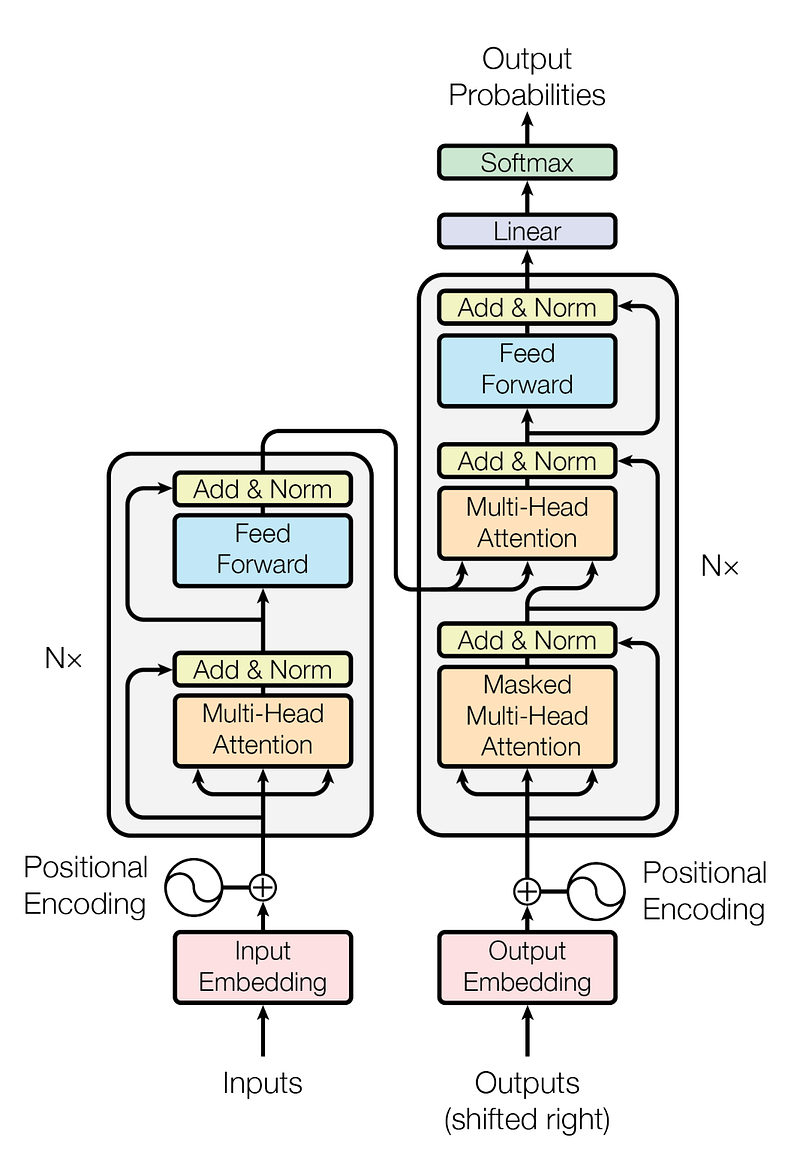Transformer architecture diagram from Attention is All You Need

Transformer uses stacked multi-head attention and dense layers for both the encoder and decoder. The encoder maps an input sequence of symbol representations to a sequence of continuous representations. Then the decoder takes the continuous representation and generates an output sequence of symbols one element at a time.

Positional Encoding

Since Transformer doesn’t contain any recurrence or convolution, positional encoding is added to give the model some information about the relative position of the words in the sentence.The formula for calculating the positional encoding

The positional encoding vector is added to the embedding vector. Embeddings represent a token in a d-dimensional space where tokens with similar meaning will be closer to each other. But the embeddings do not encode the relative position of words in a sentence. So after adding the positional encoding, words will be closer to each other based on the similarity of their meaning and their position in the sentence, in the d-dimensional space. To learn more about Positional Encoding, check out this tutorial.

We implemented the Positional Encoding with Model subclassing where we apply the encoding matrix to the input in `call()`.

``````class PositionalEncoding(tf.keras.layers.Layer):

def __init__(self, position, d_model):
super(PositionalEncoding, self).__init__()
self.pos_encoding = self.positional_encoding(position, d_model)

def get_angles(self, position, i, d_model):
angles = 1 / tf.pow(10000, (2 * (i // 2)) / tf.cast(d_model, tf.float32))
return position * angles

def positional_encoding(self, position, d_model):
position=tf.range(position, dtype=tf.float32)[:, tf.newaxis],
i=tf.range(d_model, dtype=tf.float32)[tf.newaxis, :],
d_model=d_model)
# apply sin to even index in the array
# apply cos to odd index in the array

pos_encoding = tf.concat([sines, cosines], axis=-1)
pos_encoding = pos_encoding[tf.newaxis, ...]
return tf.cast(pos_encoding, tf.float32)

def call(self, inputs):
return inputs + self.pos_encoding[:, :tf.shape(inputs), :]

``````

Implementation of Positional Encoding with Model subclassing

Transformer with Functional API

With the Functional API, we can stack our layers similar to Sequential model but without the constraint of it being a sequential model, and without declaring all the variables and layers we needed in advance like Model subclassing. One advantage of the Functional API is that it validate the model as we build it, such as checking the input and output shape for each layer, and raise meaningful error message when there is a mismatch.

We are implementing our encoding layers, encoder, decoding layers, decoder and the Transformer itself using the Functional API.

Checkout how to implement the same models with Model subclassing from this tutorial.

Encoding Layer

Each encoder layer consists of sublayers:

• 2 dense layers followed by dropout
``````def encoder_layer(units, d_model, num_heads, dropout, name="encoder_layer"):
inputs = tf.keras.Input(shape=(None, d_model), name="inputs")

'query': inputs,
'key': inputs,
'value': inputs,
})
attention = tf.keras.layers.Dropout(rate=dropout)(attention)
attention = tf.keras.layers.LayerNormalization(
epsilon=1e-6)(inputs + attention)

outputs = tf.keras.layers.Dense(units=units, activation='relu')(attention)
outputs = tf.keras.layers.Dense(units=d_model)(outputs)
outputs = tf.keras.layers.Dropout(rate=dropout)(outputs)
outputs = tf.keras.layers.LayerNormalization(
epsilon=1e-6)(attention + outputs)

return tf.keras.Model(

``````

Implementation of an encoder layer with Functional API

We can use `tf.keras.utils.plot_model()` to visualize our model. (Checkout all the model plots on the colab notebook)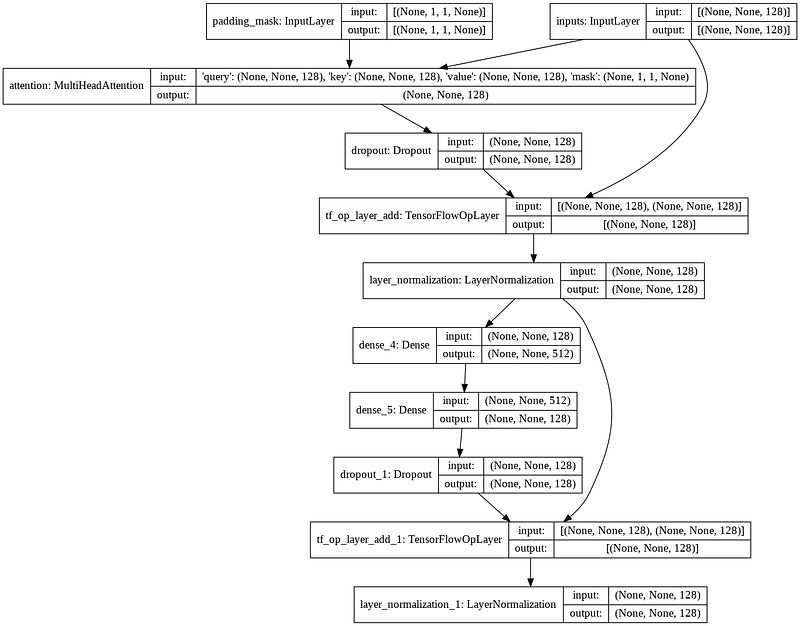Flow diagram of an encoder layer

Encoder

The Encoder consists of:

• Input Embedding
• Positional Encoding
• N of encoder layers

The input is put through an embedding which is summed with the positional encoding. The output of this summation is the input to the encoder layers. The output of the encoder is the input to the decoder.

``````def encoder(vocab_size,
num_layers,
units,
d_model,
dropout,
name="encoder"):
inputs = tf.keras.Input(shape=(None,), name="inputs")

embeddings = tf.keras.layers.Embedding(vocab_size, d_model)(inputs)
embeddings *= tf.math.sqrt(tf.cast(d_model, tf.float32))
embeddings = PositionalEncoding(vocab_size, d_model)(embeddings)

outputs = tf.keras.layers.Dropout(rate=dropout)(embeddings)

for i in range(num_layers):
outputs = encoder_layer(
units=units,
d_model=d_model,
dropout=dropout,
name="encoder_layer_{}".format(i),

return tf.keras.Model(

``````

Implementation of encoder with Functional API

Decoder Layer

Each decoder layer consists of sublayers:

• 2 dense layers followed by dropout

As query receives the output from decoder’s first attention block, and key receives the encoder output, the attention weights represent the importance given to the decoder’s input based on the encoder’s output. In other words, the decoder predicts the next word by looking at the encoder output and self-attending to its own output.

``````def decoder_layer(units, d_model, num_heads, dropout, name="decoder_layer"):
inputs = tf.keras.Input(shape=(None, d_model), name="inputs")
enc_outputs = tf.keras.Input(shape=(None, d_model), name="encoder_outputs")

'query': inputs,
'key': inputs,
'value': inputs,
})
attention1 = tf.keras.layers.LayerNormalization(
epsilon=1e-6)(attention1 + inputs)

'query': attention1,
'key': enc_outputs,
'value': enc_outputs,
})
attention2 = tf.keras.layers.Dropout(rate=dropout)(attention2)
attention2 = tf.keras.layers.LayerNormalization(
epsilon=1e-6)(attention2 + attention1)

outputs = tf.keras.layers.Dense(units=units, activation='relu')(attention2)
outputs = tf.keras.layers.Dense(units=d_model)(outputs)
outputs = tf.keras.layers.Dropout(rate=dropout)(outputs)
outputs = tf.keras.layers.LayerNormalization(
epsilon=1e-6)(outputs + attention2)

return tf.keras.Model(
outputs=outputs,
name=name)

``````

Implementation of decoder layer with Functional API

Decoder

The Decoder consists of:

• Output Embedding
• Positional Encoding
• N decoder layers

The target is put through an embedding which is summed with the positional encoding. The output of this summation is the input to the decoder layers. The output of the decoder is the input to the final linear layer.

``````def decoder(vocab_size,
num_layers,
units,
d_model,
dropout,
name='decoder'):
inputs = tf.keras.Input(shape=(None,), name='inputs')
enc_outputs = tf.keras.Input(shape=(None, d_model), name='encoder_outputs')

embeddings = tf.keras.layers.Embedding(vocab_size, d_model)(inputs)
embeddings *= tf.math.sqrt(tf.cast(d_model, tf.float32))
embeddings = PositionalEncoding(vocab_size, d_model)(embeddings)

outputs = tf.keras.layers.Dropout(rate=dropout)(embeddings)

for i in range(num_layers):
outputs = decoder_layer(
units=units,
d_model=d_model,
dropout=dropout,
name='decoder_layer_{}'.format(i),

return tf.keras.Model(
outputs=outputs,
name=name)

``````

Implementation of a decoder with Functional API

Transformer

Transformer consists of the encoder, decoder and a final linear layer. The output of the decoder is the input to the linear layer and its output is returned.

`enc<em>padding</em>mask` and `dec_padding_mask` are used to mask out all the padding tokens. `look_ahead_mask` is used to mask out future tokens in a sequence. As the length of the masks changes with different input sequence length, we are creating these masks with Lambda layers.

``````def transformer(vocab_size,
num_layers,
units,
d_model,
dropout,
name="transformer"):
inputs = tf.keras.Input(shape=(None,), name="inputs")
dec_inputs = tf.keras.Input(shape=(None,), name="dec_inputs")

# mask the future tokens for decoder inputs at the 1st attention block
output_shape=(1, None, None),
# mask the encoder outputs for the 2nd attention block

enc_outputs = encoder(
vocab_size=vocab_size,
num_layers=num_layers,
units=units,
d_model=d_model,
dropout=dropout,

dec_outputs = decoder(
vocab_size=vocab_size,
num_layers=num_layers,
units=units,
d_model=d_model,
dropout=dropout,

outputs = tf.keras.layers.Dense(units=vocab_size, name="outputs")(dec_outputs)

return tf.keras.Model(inputs=[inputs, dec_inputs], outputs=outputs, name=name)

``````

Implementation of Transformer with Functional API

Train the model

We can initialize our Transformer as follows:

``````NUM_LAYERS = 2
D_MODEL = 256
UNITS = 512
DROPOUT = 0.1

model = transformer(
vocab_size=VOCAB_SIZE,
num_layers=NUM_LAYERS,
units=UNITS,
d_model=D_MODEL,
dropout=DROPOUT)

``````

After defining our loss function, optimizer and metrics, we can simply train our model with `model.fit()`. Notice that we have to mask our loss function such that the padding tokens get ignored, also we are writing our custom learning rate.

``````def loss_function(y_true, y_pred):
y_true = tf.reshape(y_true, shape=(-1, MAX_LENGTH - 1))

loss = tf.keras.losses.SparseCategoricalCrossentropy(
from_logits=True, reduction='none')(y_true, y_pred)

return tf.reduce_mean(loss)

class CustomSchedule(tf.keras.optimizers.schedules.LearningRateSchedule):

def __init__(self, d_model, warmup_steps=4000):
super(CustomSchedule, self).__init__()

self.d_model = d_model
self.d_model = tf.cast(self.d_model, tf.float32)

self.warmup_steps = warmup_steps

def __call__(self, step):
arg1 = tf.math.rsqrt(step)
arg2 = step * (self.warmup_steps**-1.5)

return tf.math.rsqrt(self.d_model) * tf.math.minimum(arg1, arg2)

learning_rate = CustomSchedule(D_MODEL)

learning_rate, beta_1=0.9, beta_2=0.98, epsilon=1e-9)

def accuracy(y_true, y_pred):
# ensure labels have shape (batch_size, MAX_LENGTH - 1)
y_true = tf.reshape(y_true, shape=(-1, MAX_LENGTH - 1))
accuracy = tf.metrics.SparseCategoricalAccuracy()(y_true, y_pred)
return accuracy

model.compile(optimizer=optimizer, loss=loss_function, metrics=[accuracy])

EPOCHS = 20

model.fit(dataset, epochs=EPOCHS)

``````
Evaluation

To evaluate, we have to run inference one time-step at a time, and pass in the output from the previous time-step as input.

Notice that we don’t normally apply dropout during inference, but we didn’t specify a `training` argument for our model. This is because `training` and `mask` are already built-in for us, if we want to run `model` for evaluation, we can simply call `model(inputs, training=False)` to run the model in inference mode.

``````def evaluate(sentence):
sentence = preprocess_sentence(sentence)

sentence = tf.expand_dims(
START_TOKEN + tokenizer.encode(sentence) + END_TOKEN, axis=0)

output = tf.expand_dims(START_TOKEN, 0)

for i in range(MAX_LENGTH):
predictions = model(inputs=[sentence, output], training=False)

# select the last word from the seq_len dimension
predictions = predictions[:, -1:, :]
predicted_id = tf.cast(tf.argmax(predictions, axis=-1), tf.int32)

# return the result if the predicted_id is equal to the end token
if tf.equal(predicted_id, END_TOKEN):
break

# concatenated the predicted_id to the output which is given to the decoder as its input.
output = tf.concat([output, predicted_id], axis=-1)

return tf.squeeze(output, axis=0)

def predict(sentence):
prediction = evaluate(sentence)
predicted_sentence = tokenizer.decode([i for i in prediction if i < tokenizer.vocab_size])
return predicted_sentence

``````

Transformer evaluation implementation

To test our model, we can call `predict(sentence)`.

``````>>> output = predict(‘Where have you been?’)
>>> print(output)
i don t know . i m not sure . i m a paleontologist .

``````
Summary

Here we are, we have implemented a Transformer in TensorFlow 2.0 in around 500 lines of code.

In this tutorial, we focus on the two different approaches to implement complex models with Functional API and Model subclassing, and how to incorporate them.

If you want to know more about the two different approaches and their pros and cons, check out when to use the functional API section on TensorFlow’s guide.

Try using a different dataset or hyper-parameters to train the Transformer!